### The Last Stand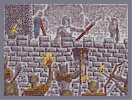Hover over the thumbnail for a full-size version.

Author RedSham author:redsham n-art nart nonplayable rated 2008-10-15 2008-10-15 5 by 94 people. \$The Last Stand#RedSham#none#00000000000000000000000000000000000000000000000000000000000000000000000000000000000000000000000000000000000000000000000000000000000000000000000000000000000000000000000000000000000000000000000000000000000000000000000000000000000000000000000000000000000000000000000000000000000000000000000000000000000000000000000000000000000000000000000000000000000000000000000000000000000000000000000000000000000000000000000000000000000000000000000000000000000000000000000000000000000000000000000000000000000000000000000000000000000000000000000000000000000000000000000000000000000000000000000000000000000000000000000000000000000000000000000000000000000000000000000000000000000000000000000000000000000000000000000000000000000000000|8^378,564,2!8^378,558,2!8^384,552,2!8^384,564,2!8^390,546,2!8^390,564,2!8^420,564,0!8^420,558,0!8^414,564,0!8^414,552,0!8^408,564,0!8^408,546,0!8^396,546,3!8^402,546,3!8^402,552,3!8^396,552,3!8^396,558,3!8^402,558,3!8^402,564,3!8^396,564,3!8^396,570,3!8^402,570,3!8^402,576,3!8^396,576,3!0^378,546!0^378,558!0^372,552!0^384,552!0^384,540!0^390,546!0^396,540!0^402,546!0^408,540!0^396,552!0^408,552!0^414,546!0^414,558!0^420,552!0^420,564!0^426,558!0^426,570!0^414,570!0^390,558!0^402,558!0^396,564!0^402,570!0^408,564!0^390,570!0^384,564!0^378,570!0^372,564!10^389,541!10^381,543!10^379,546!10^375,549!10^373,551!10^371,556!10^371,561!10^370,564!10^371,568!10^371,572!3^410,540!3^416,543!3^421,550!3^427,556!3^428,563!3^430,569!3^377,570!3^377,564!3^379,557!3^381,552!3^386,548!3^411,544!3^415,547!3^420,555!3^422,566!3^425,572!3^397,539!3^404,539!3^387,570!3^388,559!3^389,552!3^394,549!3^401,551!3^411,559!3^412,568!3^396,567!3^393,557!3^405,569!3^393,540!10^356,538!10^359,546!10^361,553!10^362,558!10^362,565!10^362,569!3^360,540!3^363,546!3^365,551!3^438,534!3^437,542!3^438,549!3^437,556!3^437,563!3^432,546!3^438,571!8^306,438,0!8^300,438,0!8^306,450,0!8^300,450,0!8^294,444,0!0^312,432!0^306,438!0^312,444!0^300,444!0^306,450!0^312,456!0^300,456!0^300,432!10^300,434!10^296,438!10^296,441!10^295,445!10^296,451!10^298,455!3^305,434!3^301,438!3^300,445!3^312,433!3^315,443!3^314,450!3^305,457!8^300,468,3!8^300,474,3!8^300,480,3!8^294,480,3!8^294,486,3!8^300,486,3!8^294,492,3!8^288,498,3!8^294,498,3!8^282,504,3!8^294,504,3!8^282,516,1!8^294,516,1!8^282,510,2!8^294,510,0!8^288,514,1!8^288,508,3!8^286,511,2!8^288,511,0!11^12,12,288,510!11^12,12,288,516!0^294,462!0^306,462!0^300,468!0^306,474!0^294,474!0^288,480!0^300,480!0^294,486!0^300,492!0^288,492!0^294,498!0^282,498!0^276,504!0^288,504!0^282,510!0^294,510!0^288,516!0^294,522!0^282,522!0^300,516!0^276,516!0^300,504!10^297,464!10^294,470!10^293,472!10^289,476!10^287,481!10^286,488!10^289,493!10^283,497!10^280,499!10^275,506!3^299,464!3^295,469!3^293,478!3^281,508!3^286,501!3^306,467!3^305,478!3^302,484!3^300,494!3^301,500!3^301,507!3^298,515!3^295,520!12^296,388!12^296,393!12^294,396!12^287,393!12^292,395!12^287,397!12^291,401!12^294,406!12^297,415!12^299,418!12^299,424!12^299,429!12^300,431!12^302,437!12^299,387!12^304,389!12^304,398!12^307,403!12^306,406!12^305,411!12^304,418!12^305,423!12^306,427!12^307,433!12^305,437!12^314,460!12^315,466!12^315,471!12^315,475!12^316,480!12^314,477!12^294,402!12^300,402!12^300,396!12^300,390!12^306,396!12^294,396!12^300,408!12^300,408!12^300,414!0^300,396!0^300,408!0^300,420!0^300,432!0^288,396!0^300,384!10^295,406!10^298,414!10^298,428!10^298,418!10^298,430!3^302,404!3^302,415!12^279,392!12^279,387!12^279,383!12^283,376!12^283,369!12^278,356!12^279,358!12^285,366!12^290,366!12^297,360!12^297,352!12^301,339!12^300,339!12^298,348!12^298,358!12^301,362!12^307,366!12^310,359!12^312,360!12^309,368!12^310,378!12^283,387!12^287,378!12^291,371!12^297,367!12^301,367!12^307,371!12^308,376!12^309,382!12^284,381!12^285,377!12^288,371!12^280,359!12^281,363!12^284,371!12^285,378!12^291,366!12^295,361!12^299,352!12^300,345!12^310,361!12^307,365!12^308,374!0^288,366!0^294,360!0^300,354!0^300,342!0^300,366!0^306,360!0^306,372!0^312,384!0^282,390!0^288,384!0^282,378!10^292,392!10^289,396!10^295,400!3^298,389!3^301,394!0^286,359!0^283,354!0^282,348!0^277,351!0^279,372!0^279,364!0^278,351!0^279,340!0^282,338!0^285,349!0^288,351!0^291,337!0^292,329!0^296,321!0^298,330!0^291,350!0^293,342!0^274,377!0^274,367!0^273,359!0^307,353!0^309,348!0^313,357!0^311,371!12^288,366!12^294,360!12^300,360!12^300,366!12^312,372!12^282,378!12^306,354!11^12,12,300,378!11^12,12,300,372!11^12,12,288,378!12^286,380!12^289,375!12^304,369!12^283,377!12^294,378!12^306,372!12^234,515!12^239,516!12^243,511!12^248,510!12^252,508!12^256,506!12^259,506!12^264,506!12^269,507!12^273,510!12^278,512!12^286,517!12^292,521!12^292,526!12^291,530!12^289,534!12^285,540!12^282,545!12^274,551!12^272,556!12^269,560!12^233,516!12^238,514!12^189,528!12^193,529!12^202,533!12^207,534!12^210,532!12^214,529!12^217,525!12^218,523!12^220,522!12^224,521!12^226,519!12^182,531!12^180,531!12^178,531!12^172,533!12^168,534!12^163,537!12^157,538!12^153,540!12^146,542!12^142,545!12^143,551!12^147,557!12^148,561!12^153,566!12^156,571!12^264,510!12^264,516!12^270,516!12^270,510!12^276,516!12^276,522!12^282,522!12^282,516!12^288,522!12^288,528!12^282,528!12^258,516!12^258,510!12^252,510!12^252,516!12^246,516!12^240,516!12^228,522!12^234,522!12^240,522!12^246,522!12^252,522!12^252,522!12^258,522!12^264,522!12^270,522!12^270,522!12^270,528!12^276,528!12^276,534!12^282,534!12^288,534!12^150,546!12^144,546!12^144,552!12^150,552!12^156,552!12^156,546!12^162,546!12^162,552!12^162,540!12^168,540!12^168,546!12^156,540!12^174,546!12^174,540!12^174,534!12^180,534!12^186,534!12^192,534!12^198,534!12^180,540!12^186,540!12^192,540!12^198,540!12^204,540!12^210,540!12^216,540!12^216,534!12^210,534!12^222,528!12^216,528!12^222,534!12^222,540!12^228,540!12^228,534!12^228,528!12^234,528!12^234,534!12^234,540!12^240,534!12^240,540!12^240,528!12^246,528!12^246,534!12^246,540!12^252,540!12^252,534!12^252,528!12^258,528!12^258,534!12^258,540!12^264,540!12^264,534!12^264,534!12^258,528!12^264,528!12^270,534!12^270,540!12^270,546!12^276,546!12^276,540!12^282,540!12^150,558!12^162,558!12^162,558!12^156,558!12^168,558!12^168,552!12^174,552!12^174,558!12^156,564!12^162,564!12^168,564!12^174,564!12^174,570!12^168,570!12^162,570!12^162,570!12^180,570!12^180,564!12^180,558!12^180,552!12^180,546!12^186,552!12^186,546!12^192,558!12^186,558!12^186,564!12^186,564!12^186,570!12^192,564!12^192,570!12^198,564!12^198,570!12^204,570!12^204,564!12^210,564!12^210,570!12^216,570!12^216,564!12^222,564!12^222,570!12^228,564!12^228,570!12^234,570!12^234,564!12^240,564!12^240,570!12^240,570!12^246,564!12^246,570!12^252,570!12^252,564!12^258,564!12^258,570!12^266,566!12^262,570!12^264,564!12^264,558!12^264,552!12^264,546!12^270,552!12^258,546!12^258,552!12^258,558!12^252,558!12^252,552!12^252,546!12^246,546!12^252,552!12^246,552!12^246,558!12^240,552!12^240,558!12^240,546!12^234,552!12^234,558!12^234,546!12^228,546!12^228,552!12^228,558!12^222,558!12^222,552!12^222,546!12^192,546!12^192,552!12^198,558!12^198,552!12^198,546!12^204,552!12^204,552!12^204,552!12^204,558!12^204,546!12^210,546!12^210,552!12^210,558!12^216,552!12^216,558!12^216,546!0^252,516!0^264,516!0^264,528!0^252,528!0^276,528!0^276,516!0^288,528!0^240,516!0^252,504!0^264,504!0^240,528!0^240,540!0^252,540!0^264,540!0^276,540!0^264,552!0^264,564!0^252,552!0^252,564!0^240,564!0^240,552!0^228,552!0^228,540!0^228,528!0^216,540!0^228,516!0^216,528!0^204,540!0^192,540!0^192,528!0^180,528!0^180,540!0^168,540!0^156,540!0^156,552!0^144,552!0^156,564!0^168,564!0^168,552!0^180,552!0^180,564!0^192,564!0^192,552!0^204,552!0^204,564!0^216,564!0^216,552!0^228,564!10^153,555!10^159,550!10^168,548!10^176,548!10^187,549!10^197,548!10^207,543!10^217,538!10^232,530!10^248,528!10^160,558!10^159,562!10^163,567!10^173,562!10^167,558!10^182,565!10^185,558!10^180,555!10^193,557!10^193,563!10^201,563!10^202,555!10^207,548!10^212,557!10^217,562!10^223,557!10^234,546!10^232,535!10^246,536!10^232,543!10^223,549!10^235,563!10^243,553!10^246,562!10^252,562!10^248,551!10^253,522!10^263,520!10^274,533!10^259,535!10^266,548!10^264,556!8^204,522,1!8^204,516,1!8^192,516,1!8^198,510,1!8^204,504,1!8^210,504,1!8^204,498,1!8^192,498,1!8^186,492,1!8^204,492,1!8^216,492,1!8^186,486,1!8^204,486,1!8^216,486,1!8^186,480,2!8^216,480,0!8^192,480,2!8^210,480,0!8^198,480,2!8^204,480,0!8^186,468,2!8^192,468,2!8^198,468,2!8^216,468,0!8^210,468,0!8^192,462,2!8^210,462,0!8^198,462,2!8^204,462,0!8^204,474,0!8^202,461,3!8^201,465,3!8^201,470,3!8^201,478,1!8^201,473,1!0^192,456!0^204,456!0^216,456!0^210,462!0^198,462!0^186,462!0^180,468!0^192,468!0^204,468!0^216,468!0^222,474!0^222,462!0^216,480!0^222,486!0^216,492!0^210,510!0^216,516!0^210,522!0^204,528!0^198,522!0^186,522!0^192,516!0^204,516!0^198,510!0^192,504!0^204,504!0^186,498!0^180,492!0^180,480!0^186,474!0^186,486!0^192,480!0^192,492!0^198,498!0^210,498!0^204,492!0^198,486!0^210,486!0^210,474!0^204,480!0^198,474!10^188,458!10^185,461!10^183,467!10^182,474!10^180,481!10^180,488!10^183,489!10^184,495!10^191,501!10^195,504!10^195,509!10^194,514!10^192,518!10^193,524!10^200,525!10^181,463!10^178,474!10^193,454!3^205,454!3^213,456!3^220,464!3^222,475!3^223,483!3^220,494!3^213,508!3^216,516!3^200,518!3^201,509!3^194,465!3^188,471!3^186,476!3^187,485!3^190,492!3^196,497!3^200,500!3^201,470!3^194,477!3^196,490!3^205,498!3^211,491!3^213,479!3^208,465!3^206,487!3^211,523!10^168,448!10^169,456!10^170,459!10^171,465!10^173,475!10^173,479!10^173,486!3^225,453!3^225,462!3^224,470!3^227,478!3^228,479!3^177,460!3^174,454!3^226,454!3^228,468!3^231,476!3^227,444!3^229,483!3^230,488!3^172,494!3^173,492!8^144,564,3!8^144,570,3!8^144,576,3!8^138,570,3!8^138,576,3!0^138,558!0^144,564!0^150,558!0^150,570!0^138,570!0^132,564!12^143,555!12^150,557!12^153,563!12^153,565!10^144,556!10^150,557!10^153,561!10^154,571!10^136,560!10^135,567!10^134,572!3^139,565!3^141,573!2^480,516,-1,0!2^479,522,-1,0!2^477,539,-1,0!2^477,549,-1,0!2^476,560,-1,0!2^474,568,-1,0!2^478,529,-1,0!2^479,524,1,0!2^480,529,1,0!2^481,536,1,0!2^481,543,1,0!2^481,551,1,0!2^479,561,1,0!2^477,569,1,0!2^473,571,1,0!2^476,561,1,0!2^478,549,1,0!2^479,541,1,0!2^480,534,1,0!3^478,520!3^478,529!3^476,540!3^482,551!3^481,564!3^471,567!3^474,556!3^474,549!12^690,412!12^687,420!12^682,427!12^679,433!12^677,439!12^664,463!12^660,470!12^655,478!12^652,484!8^666,450,2!8^672,450,2!8^672,456,2!8^678,450,2!8^678,456,2!8^684,456,3!8^690,462,2!8^696,462,2!8^702,468,2!8^708,468,2!8^714,468,2!8^714,474,2!8^720,474,2!8^720,468,2!8^726,474,2!8^726,480,2!8^732,480,2!8^738,480,2!8^744,480,3!8^753,484,2!0^666,450!0^672,444!0^660,444!0^660,456!0^672,456!0^666,462!0^678,462!0^684,456!0^678,450!0^690,462!0^684,468!0^696,468!0^696,456!0^702,462!0^714,462!0^708,468!0^702,474!0^714,474!0^726,474!0^720,480!0^708,480!0^732,480!0^738,474!0^744,492!0^744,480!0^750,474!10^663,445!10^661,451!10^662,458!10^668,462!10^673,463!10^678,463!10^682,461!10^687,468!10^693,472!10^700,475!10^705,479!10^711,482!10^722,484!10^725,479!3^672,448!3^670,457!3^688,458!3^701,468!3^710,473!3^701,459!3^710,460!3^718,463!3^724,470!3^727,471!3^736,472!3^744,474!3^730,481!3^679,451!12^693,404!12^693,400!12^700,391!12^708,389!12^714,387!12^719,384!12^730,379!12^736,377!12^744,372!12^752,366!12^646,492!12^641,499!12^631,568!12^631,575!12^696,395!12^725,384!12^741,375!12^749,368!12^643,495!12^648,489!12^658,475!12^685,423!12^689,417!12^692,406!12^638,501!12^636,506!12^631,512!12^626,516!12^626,520!12^628,528!12^631,536!12^630,543!12^630,552!12^631,560!12^631,567!12^629,549!12^630,541!12^629,534!12^628,525!12^675,443!12^676,445!12^690,420!12^691,415!12^692,409!12^694,404!12^695,402!12^700,397!12^704,396!12^679,444!12^681,438!12^683,434!12^685,430!12^687,427!12^690,424!12^695,422!12^696,420!12^697,415!12^698,411!12^698,407!12^699,402!12^701,399!12^702,396!12^708,394!12^711,391!12^714,390!12^717,389!12^721,387!12^722,387!12^728,384!12^666,471!12^662,472!12^661,475!12^658,481!12^656,486!12^654,491!12^653,495!12^651,496!12^645,499!12^641,504!12^637,509!12^634,515!12^633,521!12^633,528!12^633,535!12^634,541!12^635,549!12^634,554!12^634,559!12^633,566!12^632,547!12^628,521!12^631,517!12^646,496!2^745,381,0.707106781186547,0.707106781186547!2^640,564,0.707106781186547,0.707106781186547!0^636,504!0^648,492!0^660,480!0^684,432!0^696,420!0^696,408!0^696,396!0^708,396!0^720,384!0^744,372!0^732,384!0^624,516!0^636,516!0^636,528!0^636,540!0^636,552!0^636,564!10^678,436!10^682,428!10^687,420!10^691,414!10^693,407!10^695,400!10^703,391!10^712,389!10^717,388!10^723,386!10^728,382!10^736,374!10^742,369!10^632,572!10^633,561!10^634,549!10^633,541!10^633,533!10^633,524!10^631,519!10^636,508!10^641,504!10^645,498!10^649,491!10^651,484!10^656,478!10^659,474!10^660,473!0^714,486!0^726,486!0^744,492!0^756,480!3^715,487!3^721,485!3^731,486!3^685,452!10^735,487!10^738,489!10^744,492!10^749,492!10^756,490!3^740,481!3^748,487!3^749,477!3^758,480!12^752,494!12^755,490!12^766,484!8^720,528,2!8^726,534,2!8^732,540,2!8^732,534,2!8^738,546,2!8^738,540,2!8^744,540,0!8^744,546,0!8^742,541,1!8^741,539,3!11^12,12,738,540!11^12,12,744,540!11^12,12,744,546!0^708,522!0^720,522!0^726,540!0^726,528!0^732,534!0^744,534!0^738,540!0^750,540!0^732,546!0^720,534!0^714,528!0^744,546!10^713,526!10^719,531!10^722,535!10^726,539!10^728,545!10^733,549!10^734,551!10^742,551!10^744,551!10^750,552!3^716,524!3^727,535!3^734,540!3^742,544!3^748,544!3^723,523!3^736,528!3^747,531!10^695,511!10^699,517!10^701,520!10^705,522!10^697,507!10^697,505!3^697,495!3^699,499!3^700,494!3^708,499!3^710,505!3^714,511!3^720,515!3^706,515!3^704,509!2^652,551,0.707106781186547,0.707106781186547!2^665,538,0.707106781186547,0.707106781186547!2^674,524,0.707106781186547,0.707106781186547!2^685,512,0.707106781186547,0.707106781186547!2^740,396,0.707106781186547,0.707106781186547!2^736,408,0.707106781186547,0.707106781186547!2^732,423,0.707106781186547,0.707106781186547!2^725,439,0.707106781186547,0.707106781186547!2^719,453,0.707106781186547,0.707106781186547!2^714,467,0.707106781186547,0.707106781186547!2^709,485,0.707106781186547,0.707106781186547!12^752,498!12^749,504!12^750,522!12^753,520!12^757,519!12^761,519!12^748,518!12^762,492!12^762,498!12^762,504!12^762,510!12^762,516!12^768,504!12^768,498!12^768,492!12^768,486!12^756,516!12^756,510!12^756,504!12^756,498!12^156,576!12^162,576!12^168,576!12^174,576!12^180,576!12^186,576!12^192,576!12^198,576!12^198,576!12^204,576!12^210,576!12^222,576!12^216,576!12^222,576!12^234,570!12^234,576!12^228,576!12^240,576!12^246,576!12^252,576!12^264,576!12^258,576!10^159,577!10^166,576!10^176,575!10^185,575!10^193,574!10^196,574!10^207,572!10^213,574!10^220,574!10^225,573!10^230,572!10^236,573!10^242,573!10^252,572!10^256,572!10^259,572!8^762,534,2!8^768,534,2!8^762,540,2!8^768,534,2!12^768,516!12^768,522!0^756,528!0^768,528!0^762,534!0^756,540!0^762,546!0^768,540!10^757,550!10^765,546!10^767,543!10^769,539!10^748,533!10^753,536!3^756,529!3^766,528!3^769,537!3^760,547!3^761,537!12^752,499!12^750,504!12^749,507!12^748,512!12^747,515!12^746,521!12^750,559!12^749,564!12^754,559!12^755,559!12^751,515!0^756,504!0^768,504!0^768,492!0^756,492!0^756,516!0^768,516!0^744,516!10^754,498!10^750,501!10^749,507!10^747,514!10^747,519!10^755,520!10^753,513!10^754,504!10^754,560!10^763,493!10^759,509!10^766,498!10^764,498!10^758,494!10^758,489!10^760,482!10^762,478!3^768,487!3^765,477!3^763,505!3^639,566!3^646,561!3^653,551!3^658,547!3^666,540!3^671,532!3^675,526!3^680,519!3^689,509!3^746,383!3^743,394!3^739,404!3^736,414!3^733,425!3^729,435!3^726,445!3^721,455!3^718,465!3^714,475!3^711,485!12^652,463!12^659,468!12^667,473!12^674,478!12^681,482!12^688,486!12^693,489!3^653,465!3^662,470!3^668,474!3^675,479!3^681,483!3^691,489!12^644,459!12^636,453!12^630,448!12^622,444!12^614,439!2^611,435,-0.707106781186547,0.707106781186547!3^614,440!3^622,444!3^630,449!3^638,456!3^644,459!12^608,428!12^611,424!12^613,418!12^614,429!12^614,410!0^605,422!0^608,414!0^616,398!0^617,409!0^616,423!0^615,417!0^612,405!12^615,417!8^84,570,0!8^84,558,0!8^84,546,0!8^78,546,0!8^78,558,0!8^78,570,0!8^78,534,0!8^72,528,0!8^72,546,0!8^72,558,0!8^66,522,0!8^66,534,0!8^66,546,0!8^66,558,0!8^60,516,0!8^60,534,0!8^60,552,0!8^54,510,0!8^54,528,0!8^54,546,0!8^48,504,0!8^48,522,0!8^48,540,0!8^48,558,0!8^42,504,0!8^42,522,0!8^42,540,0!8^42,558,0!8^42,570,0!8^36,504,0!8^36,522,0!8^36,498,0!8^36,534,0!8^36,552,0!8^36,570,0!8^30,498,0!8^30,516,0!8^30,534,0!8^30,552,0!8^30,570,0!8^24,492,0!8^24,510,0!8^24,528,0!8^24,546,0!8^24,564,0!8^24,570,0!8^18,492,0!8^18,510,0!8^18,528,0!8^18,546,0!8^18,564,0!8^18,570,0!0^30,492!0^30,504!0^36,498!0^42,504!0^48,498!0^42,492!0^54,504!0^48,510!0^60,510!0^54,516!0^66,516!0^60,522!0^72,522!0^78,528!0^84,534!0^84,546!0^90,540!0^90,552!0^84,558!0^90,564!0^84,570!0^90,576!0^78,564!0^72,558!0^60,558!0^66,552!0^78,552!0^78,540!0^72,546!0^72,534!0^66,528!0^66,540!0^36,510!0^42,516!0^30,516!0^36,522!0^48,522!0^54,528!0^60,534!0^42,528!0^48,534!0^36,534!0^30,528!0^42,540!0^30,540!0^36,546!0^48,546!0^54,540!0^60,546!0^42,552!0^54,552!0^48,558!0^36,558!0^30,552!0^30,564!0^42,564!0^36,570!0^48,570!0^30,576!0^42,576!0^24,486!0^24,498!0^24,510!0^24,522!0^24,534!0^24,546!0^24,558!0^24,570!10^46,575!10^50,568!10^57,565!10^61,563!10^67,563!10^78,563!10^74,562!10^26,555!10^26,566!10^26,572!10^35,575!10^35,566!10^33,554!3^27,506!3^30,515!3^29,526!3^28,535!3^29,545!3^34,552!3^37,558!3^43,561!3^55,558!3^45,547!3^40,544!3^35,530!3^35,494!3^27,506!3^56,506!3^47,502!3^36,504!3^30,510!3^24,490!3^60,550!3^77,548!3^60,511!3^70,521!3^72,531!3^90,568!3^90,561!3^88,552!3^82,531!3^84,541!3^89,550!3^84,556!3^90,573!3^44,511!3^39,522!3^46,532!3^63,539!3^60,528!3^50,518!3^57,541!3^27,500!3^26,493!3^40,497!3^50,500!3^58,503!3^66,510!3^77,518!3^83,526!3^88,533!3^91,551!3^27,499!3^27,508!3^27,518!3^28,528!3^31,544!3^38,545!3^37,534!3^35,519!3^49,550!3^43,513!3^48,511!3^36,506!3^34,525!10^33,561!10^43,569!10^30,551!10^63,577!10^65,576!10^69,575!10^72,576!10^73,576!3^57,578!3^64,573!12^68,487!12^76,477!12^84,467!12^91,455!12^97,446!12^102,451!12^96,460!12^90,470!12^85,479!12^79,489!12^73,499!12^66,499!12^70,494!12^75,484!12^82,475!12^101,438!12^106,443!12^109,435!12^113,427!12^104,431!12^112,424!12^118,417!12^109,427!12^112,419!12^119,410!12^125,403!12^88,459!12^115,414!12^120,405!12^125,397!12^63,494!12^70,485!12^79,472!12^94,451!12^71,503!12^76,494!12^81,485!12^88,474!12^92,467!12^97,458!12^103,447!12^106,441!12^111,432!12^116,422!12^123,408!12^66,498!12^72,498!12^72,492!12^66,492!12^72,486!12^78,480!12^72,480!12^84,474!12^84,468!12^90,462!12^90,468!12^96,456!12^96,450!12^102,444!12^108,438!12^102,438!12^120,414!12^85,463!12^92,463!12^99,455!12^98,443!12^122,403!0^72,504!0^72,492!0^72,480!0^84,480!0^84,468!0^96,456!0^96,444!0^108,432!0^120,408!0^114,420!0^126,396!2^118,402,0.707106781186547,0.707106781186547!2^114,412,0.707106781186547,0.707106781186547!2^108,423,0.707106781186547,0.707106781186547!2^98,439,0.707106781186547,0.707106781186547!2^101,433,0.707106781186547,0.707106781186547!2^93,442,-1,0!2^96,426,-1,0!2^95,410,-1,0!2^96,406,-0.707106781186547,-0.707106781186547!2^105,401,-0.707106781186547,-0.707106781186547!2^116,391,-0.707106781186547,-0.707106781186547!2^122,385,-0.707106781186547,-0.707106781186547!2^121,388,1,0!2^121,395,1,0!2^102,427,1,0!2^102,413,1,0!2^112,414,1,0!2^116,401,1,0!3^96,411!3^103,404!3^111,395!3^120,387!3^117,399!3^109,405!3^99,417!3^107,412!3^98,432!3^99,424!3^108,419!3^116,409!2^111,452,0,-1!2^121,449,0,-1!2^117,437,0,-1!2^123,424,0,-1!2^132,438,1,0!2^134,426,1,0!2^137,413,1,0!2^134,407,-0.707106781186547,-0.707106781186547!2^138,402,-0.707106781186547,-0.707106781186547!2^138,405,1,0!2^130,441,0,1!12^125,411!12^121,419!12^116,427!12^112,434!12^108,440!12^104,449!12^118,420!3^117,440!3^126,432!3^122,420!3^133,411!10^68,498!10^73,492!10^78,486!10^81,479!10^84,474!10^88,470!10^92,463!10^95,460!10^100,452!10^103,445!10^106,440!10^109,434!10^115,425!10^119,420!10^124,410!3^87,542!3^90,559!8^84,504,2!8^90,504,2!8^90,498,2!8^96,516,2!8^90,516,2!8^96,516,2!8^96,522,2!8^102,540,2!8^102,552,2!8^102,570,2!0^78,498!0^90,498!0^84,492!0^84,504!0^78,510!0^90,510!0^84,516!0^96,516!0^90,522!0^96,528!0^96,540!0^96,552!0^102,546!0^102,558!0^96,564!0^102,570!0^96,576!10^77,506!10^83,512!10^88,520!10^90,531!10^92,539!10^95,553!10^96,562!10^95,569!3^92,486!3^94,504!3^104,544!3^105,551!3^108,568!3^95,573!3^102,574!3^83,502!3^86,500!3^97,518!3^98,533!3^93,487!3^95,502!3^99,512!3^106,564!3^109,573!3^107,558!3^102,531!3^94,494!3^29,485!3^75,460!3^80,445!3^300,378!3^300,372!1^282,444!12^480,294!12^486,294!12^486,306!12^480,300!12^486,300!12^480,306!12^480,312!12^480,312!12^486,312!12^486,318!12^486,324!12^486,330!12^480,330!12^480,342!12^480,336!12^486,336!12^486,342!12^486,354!12^486,348!12^480,354!12^480,360!12^486,360!12^486,366!12^480,366!12^480,372!12^486,372!12^486,378!12^480,378!12^480,384!12^486,384!12^486,390!12^480,390!12^480,396!12^486,396!12^486,402!12^480,402!12^480,408!12^486,408!12^486,414!12^480,414!12^480,420!12^486,420!12^486,426!12^480,426!12^480,432!12^486,432!12^486,438!12^480,438!12^480,450!12^486,444!12^480,444!12^486,450!12^486,456!12^480,456!12^480,462!12^486,462!12^474,294!12^468,294!12^462,294!12^456,294!12^450,294!12^444,294!12^492,294!12^498,294!12^504,294!12^510,294!12^516,294!12^522,294!12^528,294!12^534,294!12^438,294!12^432,294!10^442,324!10^443,333!10^445,340!10^446,348!10^531,304!10^528,309!10^524,317!10^523,327!10^523,333!12^480,348!10^436,302!10^438,309!10^441,315!12^480,318!12^480,324!10^447,305!10^453,305!10^462,305!10^468,305!10^473,305!10^495,305!10^500,305!10^508,304!10^514,305!10^520,306!10^520,309!10^518,313!10^516,318!10^516,324!10^516,329!10^518,334!10^493,309!10^499,310!10^506,311!10^514,311!10^498,315!10^497,320!10^498,326!10^497,334!10^506,321!10^446,314!10^452,315!10^459,313!10^466,313!10^469,315!10^471,317!10^470,328!10^471,335!10^471,338!10^448,340!10^453,341!10^460,337!10^463,335!10^464,330!10^457,325!10^455,328!10^455,333!10^454,333!10^453,327!10^448,322!10^464,319!10^468,321!10^444,307!10^450,308!10^456,308!10^466,308!10^463,315!10^458,316!10^507,315!10^508,318!3^447,348!3^445,361!3^441,377!3^438,389!3^447,387!3^459,381!3^465,381!3^472,381!3^450,354!3^453,349!3^458,347!3^464,345!3^468,344!3^452,358!3^451,364!3^444,380!3^453,371!3^461,351!3^463,362!3^467,372!3^473,374!3^468,351!3^473,361!3^469,351!3^524,344!3^523,355!3^525,363!3^527,370!3^528,377!3^496,383!3^505,383!3^511,384!3^522,388!3^528,394!3^526,383!3^497,344!3^494,344!3^498,338!3^500,334!3^496,352!3^506,350!3^515,346!3^515,353!3^505,358!3^496,360!3^496,373!3^501,368!3^514,361!3^506,372!3^521,381!3^511,370!3^448,334!3^446,331!3^447,322!10^448,332!10^450,326!10^438,304!10^464,327!10^472,330!10^505,328!10^502,331!10^496,329!10^494,321!10^495,314!10^523,325!10^524,318!10^523,311!10^523,306!10^526,304!10^498,346!10^462,348!10^518,351!10^509,351!10^456,357!10^471,355!10^497,357!10^475,311!10^475,317!10^476,324!10^475,331!10^474,336!10^475,342!10^476,346!10^474,324!10^445,301!10^453,301!10^457,302!10^465,301!10^471,301!10^473,301!10^492,301!10^499,302!10^505,302!10^510,301!10^512,302!0^480,294!0^486,306!0^480,318!0^486,330!0^480,342!0^486,354!0^480,366!0^486,378!0^480,390!0^486,402!0^480,414!0^486,426!0^480,438!0^486,450!0^480,462!0^462,294!0^444,294!0^504,294!0^522,294!10^477,297!10^480,304!10^481,313!10^482,321!10^480,334!10^480,347!10^480,359!10^481,367!10^480,377!10^478,387!10^480,402!10^481,415!10^481,427!10^480,441!10^480,452!10^481,461!10^435,297!10^443,299!10^453,301!10^464,299!10^478,301!10^498,299!10^507,302!10^508,300!10^520,301!8^474,432,0!8^468,432,0!8^468,438,0!8^462,444,0!8^456,450,0!8^450,450,0!8^450,456,0!8^444,456,0!8^438,456,0!8^438,462,0!8^426,456,0!8^426,462,0!0^480,426!0^474,432!0^480,438!0^468,438!0^474,444!0^462,444!0^462,456!0^468,450!0^456,450!0^444,450!0^450,456!0^438,456!0^444,462!0^456,462!0^438,468!10^482,435!10^480,440!10^476,443!10^470,443!10^469,448!10^468,452!10^466,457!10^458,461!10^464,461!10^457,464!10^453,467!10^448,470!10^440,470!10^435,470!10^427,469!10^423,454!10^423,463!3^482,420!3^476,423!3^471,424!3^467,428!3^465,430!3^462,433!3^457,434!3^455,435!3^452,440!3^451,445!3^425,451!3^432,446!3^439,444!3^444,444!3^426,462!3^432,463!3^445,464!3^449,460!3^455,457!3^430,452!3^435,457!3^455,454!3^465,451!3^469,445!3^441,458!3^457,448!3^472,437!3^481,422!3^485,421!3^492,421!3^492,427!3^490,432!3^482,436!12^428,441!12^423,442!12^419,443!12^414,443!12^409,444!12^404,445!12^397,449!12^395,450!12^391,451!12^351,425!12^367,454!12^372,458!12^376,458!12^385,457!12^389,455!12^363,452!12^403,446!12^348,478!12^352,426!12^354,427!12^359,429!12^361,435!12^361,440!12^364,445!12^367,450!12^347,427!12^346,432!12^345,436!12^344,443!12^342,450!12^341,456!12^340,460!12^341,465!12^343,469!12^346,479!12^346,483!12^347,486!12^345,475!12^348,491!12^351,492!12^356,489!12^361,486!12^431,442!12^429,446!12^425,451!12^424,454!12^422,457!12^421,461!12^420,464!12^418,469!12^417,472!12^418,478!12^417,482!12^418,485!12^418,487!12^419,490!12^368,484!12^371,481!12^381,481!12^377,482!12^363,484!12^386,482!12^389,484!12^396,491!12^402,493!12^409,494!12^412,494!12^416,496!10^426,477!10^426,482!10^424,486!10^422,491!12^348,432!12^354,432!12^354,438!12^348,438!12^360,432!12^360,438!12^360,444!12^354,444!12^348,444!12^348,450!12^354,450!12^360,450!12^360,456!12^354,456!12^354,456!12^348,456!12^342,456!12^342,462!12^348,462!12^348,468!12^348,474!12^354,462!12^354,468!12^354,474!12^354,480!12^354,486!12^348,486!12^348,486!12^348,480!12^360,480!12^360,474!12^360,468!12^360,462!12^366,462!12^366,468!12^366,474!12^366,480!12^366,456!12^372,462!12^378,462!12^384,462!12^384,462!12^396,462!12^372,468!12^372,474!12^372,480!12^378,480!12^378,474!12^378,468!12^384,468!12^384,474!12^384,480!12^390,480!12^390,474!12^390,468!12^390,462!12^390,456!12^396,456!12^402,456!12^402,450!12^408,450!12^414,450!12^420,450!12^426,444!12^420,444!12^414,444!12^420,456!12^414,456!12^408,456!12^408,462!12^402,462!12^414,462!12^414,468!12^414,474!12^414,480!12^414,486!12^414,492!12^408,492!12^402,492!12^396,486!12^402,486!12^408,486!12^408,480!12^402,480!12^396,480!12^396,474!12^408,474!12^402,474!12^408,468!12^402,468!12^396,468!12^381,458!9^756,570,0,1,32,23,0,0,0!9^756,576,0,1,32,23,0,0,0!9^762,564,0,1,32,23,0,0,0!9^762,558,0,1,32,23,0,0,0!9^768,570,0,1,32,23,0,0,0!9^768,576,0,1,32,23,0,0,0!9^768,552,0,1,32,23,0,0,0!3^758,561!3^756,567!3^758,575!3^763,572!3^761,565!0^348,444!0^360,444!0^360,432!0^348,432!0^348,456!0^360,456!0^348,468!0^348,480!0^348,492!0^360,480!0^360,468!0^372,468!0^372,456!0^384,456!0^396,456!0^408,444!0^408,456!0^420,456!0^420,444!0^432,444!0^420,468!0^408,480!0^408,492!0^396,492!0^396,480!0^384,480!0^372,480!0^384,468!0^408,468!0^396,468!10^348,454!10^348,461!10^345,471!10^348,483!10^349,487!10^354,483!10^367,478!10^371,478!10^379,478!10^383,479!10^391,482!10^394,484!10^398,487!10^402,490!10^357,470!10^353,463!10^349,450!10^364,469!10^374,469!10^384,469!10^393,464!10^392,471!10^401,478!10^408,486!10^408,475!10^407,463!10^396,463!10^351,443!10^360,483!10^358,456!10^372,465!10^411,458!10^411,464!10^416,462!10^419,455!10^406,457!2^367,423,-1,0!2^368,432,-0.707106781186547,0.707106781186547!2^372,437,0,1!2^393,439,0.707106781186547,0.707106781186547!2^364,423,-1,0!2^365,411,-1,0!2^366,406,-0.707106781186547,-0.707106781186547!2^375,397,0,-1!2^388,396,0,-1!2^396,401,0.707106781186547,-0.707106781186547!2^401,410,1,0!2^402,419,1,0!2^403,430,1,0!2^372,414,-1,0!2^372,426,-1,0!2^372,402,-1,0!2^378,402,-1,0!2^378,414,-1,0!2^378,432,-1,0!2^378,426,-1,0!2^384,402,-1,0!2^384,420,-1,0!2^384,432,-1,0!2^396,432,-1,0!2^390,432,-1,0!2^402,432,-1,0!2^402,420,-1,0!2^402,408,-1,0!2^396,408,-1,0!2^396,420,-1,0!2^390,420,-1,0!2^390,408,-1,0!2^390,402,-1,0!2^396,402,-1,0!2^384,414,-1,0!8^378,444,1!8^378,438,1!2^372,438,-1,0!2^372,438,-0.707106781186547,0.707106781186547!2^384,438,0,1!2^384,432,0,1!2^378,432,0,1!2^378,426,0,1!2^390,426,0,1!2^383,440,0,1!0^378,450!3^371,450!3^373,455!3^382,456!3^376,400!3^370,403!3^366,410!3^364,419!3^365,429!3^369,436!3^376,440!3^387,441!3^373,398!3^365,406!3^374,410!3^372,419!3^373,427!3^381,434!3^393,438!3^403,432!3^382,398!3^402,407!3^404,417!3^386,413!3^384,426!3^399,426!3^367,417!3^366,424!3^369,436!3^376,440!3^387,440!3^356,387!3^359,391!3^359,397!3^361,404!3^402,389!3^401,393!3^401,399!3^401,405!3^402,412!3^403,419!3^401,385!10^354,388!10^358,392!10^360,398!10^360,404!10^361,415!8^336,450,2!8^336,438,2!8^342,432,2!8^342,450,2!8^342,468,2!12^350,425!12^346,425!12^343,429!12^340,437!12^340,445!12^340,451!12^339,460!12^339,469!12^340,477!12^342,482!12^340,465!12^340,456!12^341,435!12^342,474!12^348,474!12^348,474!12^348,468!12^342,468!12^342,462!12^348,462!12^348,456!12^342,456!12^342,450!12^348,450!12^348,444!12^342,444!12^342,438!12^348,438!12^348,432!12^342,432!0^348,444!0^348,432!0^336,444!0^336,456!0^336,468!0^348,456!0^348,468!0^336,480!0^330,450!0^330,438!0^336,432!10^343,455!10^343,468!10^345,477!10^347,472!10^350,461!10^350,448!10^348,445!3^336,433!3^332,437!3^330,445!3^331,453!3^333,463!3^335,468!3^336,477!3^339,481!9^360,492,1,1,13,24,0,0,0!9^360,498,1,1,13,24,0,0,0!9^366,504,1,1,13,24,0,0,0!9^366,510,1,1,13,24,0,0,0!9^372,492,1,1,13,24,0,0,0!9^372,498,1,1,13,24,0,0,0!9^378,486,1,1,13,24,0,0,0!9^384,492,1,1,13,24,0,0,0!9^384,498,1,1,13,24,0,0,0!9^378,504,1,1,13,24,0,0,0!9^378,510,1,1,13,24,0,0,0!9^390,504,1,1,13,24,0,0,0!9^390,510,1,1,13,24,0,0,0!9^396,498,1,1,13,24,0,0,0!9^402,504,1,1,13,24,0,0,0!9^402,510,1,1,13,24,0,0,0!9^372,516,1,1,13,24,0,0,0!9^372,522,1,1,13,24,0,0,0!9^384,516,1,1,13,24,0,0,0!9^384,522,1,1,13,24,0,0,0!9^396,516,1,1,13,24,0,0,0!9^396,522,1,1,13,24,0,0,0!9^408,498,1,1,13,24,0,0,0!9^414,504,1,1,13,24,0,0,0!9^414,510,1,1,13,24,0,0,0!9^408,516,1,1,13,24,0,0,0!9^408,522,1,1,13,24,0,0,0!3^361,494!3^364,503!3^371,510!3^373,519!3^376,527!3^368,492!3^371,502!3^375,512!3^381,522!3^380,490!3^382,500!3^385,509!3^388,523!3^415,503!3^411,515!3^411,524!3^380,532!3^388,528!3^408,530!3^392,499!3^394,509!3^396,525!3^376,536!3^371,532!3^367,517!3^368,528!3^369,537!3^350,499!3^352,507!3^354,511!3^356,515!3^358,519!3^352,503!3^351,502!3^356,514!0^402,390!0^402,402!0^402,414!0^354,390!0^360,402!0^360,414!10^354,390!10^360,396!10^360,408!10^360,414!3^402,390!3^402,396!3^401,408!3^405,419!3^359,403!8^768,462,2!8^768,474,2!8^774,462,2!8^774,480,2!8^768,456,2!8^774,450,2!8^774,462,2!0^768,444!0^762,450!0^768,456!0^762,462!0^768,468!0^762,474!0^768,480!10^768,445!10^762,448!10^763,454!10^764,462!10^764,470!10^762,477!10^766,486!10^770,491!3^766,453!3^765,463!3^766,473!12^508,344!12^516,342!12^517,335!12^515,328!12^504,337!12^510,324!0^505,328!0^510,321!0^516,330!12^513,456!12^515,454!12^515,461!12^516,467!12^515,474!12^513,461!12^513,469!12^513,474!12^513,479!3^513,454!3^514,464!3^514,472!3^514,482!0^509,475!0^515,479!0^514,469!12^513,413!12^514,426!12^513,434!12^514,442!12^514,449!12^513,418!12^513,423!12^513,431!12^513,448!12^513,441!12^514,408!3^514,418!3^513,425!3^514,435!3^513,443!3^513,448!0^510,483!0^514,461!0^518,474!0^514,482!0^514,454!12^514,482!12^515,476!12^513,402!12^513,392!2^510,395,-1,0!2^517,395,1,0!8^540,510,2!8^540,528,2!8^546,504,2!8^546,522,2!8^546,540,2!8^552,552,2!8^552,534,2!8^552,516,2!8^552,498,2!8^582,510,0!8^582,528,0!8^576,504,0!8^576,522,0!8^576,540,0!8^570,552,0!8^570,534,0!8^570,522,0!8^570,510,0!8^570,498,0!8^564,498,0!8^558,516,2!8^564,516,0!8^558,534,2!8^564,534,0!8^558,546,2!8^564,546,0!8^558,552,2!8^564,552,0!8^558,498,2!8^561,496,3!8^560,501,3!8^560,506,3!8^559,511,3!8^559,517,3!8^560,523,3!8^560,528,3!8^560,536,3!8^560,544,3!8^560,552,3!0^558,492!0^570,492!0^564,486!0^552,486!0^552,498!0^540,498!0^546,504!0^546,492!0^564,498!0^576,498!0^582,504!0^588,510!0^540,510!0^540,522!0^534,516!0^534,504!0^534,528!0^540,534!0^546,540!0^540,546!0^546,552!0^552,546!0^552,558!0^558,552!0^564,558!0^570,552!0^576,558!0^576,546!0^582,540!0^588,534!0^588,522!0^582,516!0^582,528!0^564,546!0^558,540!0^570,540!0^576,534!0^576,522!0^576,510!0^546,528!0^552,534!0^546,516!0^552,510!0^558,504!0^570,504!0^570,516!0^570,528!0^564,534!0^552,522!0^564,522!0^558,528!0^558,516!0^564,510!10^550,489!10^542,496!10^539,506!10^537,514!10^536,527!10^538,537!10^545,545!10^549,547!10^555,548!10^562,549!10^538,504!3^550,492!3^547,499!3^541,509!3^542,524!3^543,536!3^553,544!3^568,548!3^579,545!3^579,498!3^586,508!3^585,520!3^583,535!3^555,498!3^548,511!3^549,525!3^557,539!3^571,539!3^578,526!3^557,523!3^566,533!3^575,520!3^562,492!3^557,509!3^568,523!3^579,510!10^527,490!10^531,500!10^531,509!10^530,518!10^532,531!10^528,481!10^529,492!10^533,499!10^528,529!3^589,489!3^589,501!3^589,512!3^589,521!3^592,530!3^592,483!10^550,553!10^547,559!3^553,558!3^560,558!3^574,557!12^497,565!12^502,564!12^508,562!12^516,561!12^525,559!12^538,559!12^545,561!12^559,563!12^562,563!12^569,562!12^576,561!12^580,560!12^585,559!12^591,559!12^597,559!12^602,559!12^606,560!12^611,560!12^615,560!12^618,560!12^622,561!12^498,571!12^532,559!12^626,562!12^629,564!12^626,569!12^625,572!12^553,562!12^520,560!12^513,562!12^504,570!12^510,570!12^498,576!12^504,576!12^510,576!12^516,576!12^522,576!12^528,576!12^534,576!12^540,576!12^546,576!12^552,576!12^558,576!12^564,576!12^570,576!12^576,576!12^582,576!12^588,576!12^594,576!12^600,576!12^606,576!12^612,576!12^618,576!12^624,576!12^504,564!12^510,564!12^516,564!12^516,570!12^522,570!12^522,564!12^528,564!12^528,570!12^618,570!12^618,564!12^624,564!12^624,570!12^534,564!12^534,570!12^540,570!12^540,564!12^546,564!12^552,570!12^546,570!12^552,564!12^558,564!12^558,570!12^564,570!12^564,570!12^570,564!12^564,564!12^570,570!12^576,570!12^576,564!12^582,564!12^582,570!12^588,570!12^588,564!12^594,564!12^594,570!12^600,570!12^600,564!12^606,564!12^606,570!12^612,570!12^612,564!0^516,564!0^540,564!0^528,564!0^528,576!0^516,576!0^504,576!0^504,564!0^540,576!0^552,576!0^552,564!0^564,564!0^564,576!0^576,576!0^576,564!0^588,564!0^600,564!0^612,564!0^624,564!0^624,576!0^612,576!0^600,576!0^588,576!10^499,566!10^506,574!10^508,571!10^519,573!10^535,575!10^547,578!10^563,577!10^574,575!10^587,576!10^602,577!10^529,569!10^541,569!10^559,571!10^526,577!10^572,568!10^613,575!8^498,576,2!8^630,576,0!12^499,569!12^504,575!12^504,578!12^505,569!12^627,569!12^623,573!12^623,576!10^494,571!10^493,575!3^634,571!3^636,577!0^497,577!0^630,578!3^634,577!10^496,576!8^36,372,0!8^36,378,0!8^30,384,0!8^30,396,0!8^24,396,0!8^24,384,0!8^24,372,0!8^36,366,0!8^30,366,0!8^30,360,0!8^24,366,0!8^24,354,0!8^18,354,0!8^18,372,0!8^18,390,0!8^30,402,0!8^24,402,0!8^24,408,0!8^18,408,0!8^18,402,0!8^24,432,0!8^18,426,0!8^18,432,0!0^24,348!0^30,354!0^36,360!0^42,366!0^30,366!0^24,360!0^24,372!0^36,372!0^42,378!0^30,378!0^36,384!0^24,384!0^30,390!0^36,396!0^24,396!0^30,402!0^36,408!0^24,408!0^24,420!0^24,432!0^30,426!0^30,438!3^26,347!3^32,350!3^38,357!3^41,365!3^42,375!3^39,384!3^39,394!3^40,406!3^28,418!3^35,414!3^28,426!3^32,436!3^28,357!3^35,365!3^28,381!3^37,380!3^26,391!3^30,395!3^24,411!3^24,401!3^33,405!12^42,414!12^48,413!12^55,413!12^60,418!12^60,424!12^58,433!12^60,440!12^60,446!12^60,449!12^59,456!12^57,461!12^56,466!12^52,465!12^50,464!12^44,467!12^39,469!12^32,473!12^26,437!12^29,437!12^33,432!12^33,432!12^36,423!12^36,431!12^40,420!12^31,476!12^36,470!12^48,414!12^54,414!12^54,420!12^48,420!12^42,420!12^42,426!12^48,426!12^54,426!12^60,426!12^36,426!12^42,432!12^48,432!12^54,432!12^54,438!12^54,444!12^54,450!12^54,456!12^54,462!12^60,450!12^36,438!12^42,438!12^48,438!12^48,444!12^42,444!12^36,444!12^30,444!12^24,444!12^24,450!12^24,456!12^24,462!12^24,468!12^24,474!12^30,474!12^30,468!12^30,462!12^30,456!12^30,450!12^36,450!12^36,456!12^36,462!12^36,468!12^42,462!12^42,456!12^42,450!12^48,450!12^48,456!12^48,462!12^42,468!10^24,414!10^34,409!10^27,405!3^27,426!10^36,387!10^32,387!0^31,385!0^35,383!3^31,382!0^48,420!0^48,432!0^60,420!0^60,432!0^60,444!0^60,456!0^48,456!0^48,468!0^36,468!0^24,468!0^24,480!0^24,456!0^36,456!0^36,444!0^24,444!0^36,432!0^48,444!10^26,476!10^32,469!10^39,465!10^42,464!10^52,461!10^56,461!10^28,462!10^36,455!10^46,452!10^55,453!10^26,449!10^36,444!10^47,443!10^59,443!10^54,430!8^66,438,0!8^66,456,0!8^60,426,0!8^60,444,0!8^60,462,0!8^60,474,0!0^66,420!0^66,432!0^72,438!0^66,444!0^72,450!0^66,456!0^72,462!0^66,468!12^61,419!12^60,425!12^60,431!12^59,436!12^58,446!12^56,453!12^52,465!12^56,466!12^58,441!12^58,450!12^57,458!12^57,461!12^60,432!12^60,442!12^60,449!12^60,455!12^59,461!12^54,420!12^54,420!12^60,420!12^60,426!12^54,426!12^54,432!12^60,432!12^60,438!12^54,438!12^54,444!12^54,450!12^54,456!12^54,462!0^60,432!0^60,420!0^60,444!0^60,456!10^55,461!10^59,455!10^59,449!10^57,441!10^52,428!10^50,447!3^69,424!3^73,434!3^74,443!3^73,454!9^54,474,0,1,0,19,0,-1,0!9^54,480,0,1,0,19,0,-1,0!9^42,474,0,1,0,19,0,-1,0!9^48,486,0,1,0,19,0,-1,0!9^36,486,0,1,0,19,0,-1,0!3^35,477!3^54,478!3^39,477!3^55,479!3^50,478!3^61,475!3^66,470!3^71,464!3^74,455!12^36,381!12^38,382!12^26,384!3^39,334!3^36,340!3^38,340!3^40,348!3^31,395!3^31,391!2^59,393,0,1!2^73,390,0,1!2^88,391,0,1!2^154,400,0,1!2^169,399,0,1!2^184,397,0,1!2^199,400,0,1!2^214,402,0,1!2^229,400,0,1!2^244,400,0,1!2^261,401,0,1!2^278,405,0,1!2^320,404,0,1!2^336,403,0,1!2^425,400,0,1!2^442,400,0,1!2^458,398,0,1!2^541,403,0,1!2^552,400,0,1!2^568,397,0,1!2^583,397,0,1!2^596,398,0,1!2^634,400,0,1!2^647,402,0,1!2^662,402,0,1!2^673,402,0,1!2^716,404,0,1!2^758,403,0,1!2^56,363,0,1!2^72,361,0,1!2^88,362,0,1!2^106,364,0,1!2^124,364,0,1!2^146,365,0,1!2^163,363,0,1!2^179,362,0,1!2^195,367,0,1!2^211,370,0,1!2^227,371,0,1!2^246,369,0,1!2^260,367,0,1!12^480,468!12^486,468!12^486,474!12^480,474!12^480,480!12^486,486!12^486,480!12^480,486!12^480,492!12^486,492!12^486,498!12^480,498!12^480,504!12^486,504!12^486,510!12^442,292!12^447,292!12^451,290!12^454,289!12^459,288!12^464,289!12^469,290!12^477,291!12^484,291!12^487,291!12^492,292!12^497,291!12^502,288!12^506,289!12^511,290!12^516,291!12^521,291!10^482,469!10^482,479!10^484,486!10^483,492!10^486,498!10^448,293!10^458,291!10^470,293!10^485,294!10^494,294!0^220,500!3^217,502!2^321,364,0,1!2^337,367,0,1!2^355,369,0,1!2^369,368,0,1!2^389,366,0,1!2^406,363,0,1!2^421,361,0,1!2^432,359,0,1!2^538,360,0,1!2^554,365,0,1!2^569,366,0,1!2^585,367,0,1!2^602,366,0,1!2^618,365,0,1!2^633,367,0,1!2^652,369,0,1!2^670,369,0,1!2^685,370,0,1!2^701,370,0,1!2^717,369,0,1!2^763,373,0,1!2^96,374,1,0!2^95,388,1,0!2^164,377,1,0!2^166,395,1,0!2^234,378,1,0!2^231,393,1,0!2^364,380,1,0!2^424,372,1,0!2^423,389,1,0!2^553,377,1,0!2^554,390,1,0!2^611,378,1,0!2^671,380,1,0!2^668,395,1,0!2^55,329,0,1!2^71,330,0,1!2^87,331,0,1!2^103,334,0,1!2^123,334,0,1!2^143,334,0,1!2^157,333,0,1!2^170,333,0,1!2^181,333,0,1!2^195,333,0,1!2^211,334,0,1!2^226,334,0,1!2^244,336,0,1!2^256,337,0,1!2^267,337,0,1!2^316,336,0,1!2^332,336,0,1!2^349,336,0,1!2^372,334,0,1!2^388,329,0,1!2^404,328,0,1!2^422,328,0,1!2^538,327,0,1!2^554,331,0,1!2^572,331,0,1!2^590,337,0,1!2^608,340,0,1!2^625,341,0,1!2^644,339,0,1!2^662,336,0,1!2^677,337,0,1!2^694,340,0,1!2^705,340,0,1!2^717,342,0,1!2^729,343,0,1!2^739,347,0,1!2^758,348,0,1!2^33,303,0,1!2^54,303,0,1!2^76,302,0,1!2^94,305,0,1!2^114,305,0,1!2^134,304,0,1!2^150,306,0,1!2^167,307,0,1!2^183,308,0,1!2^196,308,0,1!2^209,307,0,1!2^224,307,0,1!2^239,309,0,1!2^254,308,0,1!2^270,309,0,1!2^283,309,0,1!2^293,308,0,1!2^307,310,0,1!2^319,307,0,1!2^335,311,0,1!2^360,309,0,1!2^350,309,0,1!2^373,311,0,1!2^386,309,0,1!2^402,308,0,1!2^414,306,0,1!2^760,319,0,1!2^744,318,0,1!2^728,315,0,1!2^712,315,0,1!2^695,314,0,1!2^680,312,0,1!2^663,311,0,1!2^645,311,0,1!2^625,310,0,1!2^610,309,0,1!2^598,309,0,1!2^584,309,0,1!2^567,307,0,1!2^552,306,0,1!2^760,286,0,1!2^742,287,0,1!2^725,290,0,1!2^706,291,0,1!2^689,292,0,1!2^667,289,0,1!2^649,288,0,1!2^631,288,0,1!2^611,286,0,1!2^593,286,0,1!2^577,284,0,1!2^557,282,0,1!2^533,282,0,1!2^31,271,0,1!2^49,273,0,1!2^65,272,0,1!2^80,271,0,1!2^95,271,0,1!2^111,272,0,1!2^128,276,0,1!2^145,276,0,1!2^160,276,0,1!2^173,276,0,1!2^186,275,0,1!2^201,278,0,1!2^216,278,0,1!2^233,279,0,1!2^246,279,0,1!2^259,279,0,1!2^272,280,0,1!2^286,279,0,1!2^298,279,0,1!2^311,279,0,1!2^328,281,0,1!2^345,282,0,1!2^354,283,0,1!2^371,283,0,1!2^382,284,0,1!2^399,282,0,1!2^413,280,0,1!2^432,280,0,1!2^447,279,0,1!2^464,278,0,1!2^482,279,0,1!2^506,279,0,1!2^359,333,0,1!2^128,345,1,0!2^126,355,1,0!2^60,341,1,0!2^58,354,1,0!2^202,359,1,0!2^200,344,1,0!2^332,346,1,0!2^333,360,1,0!2^263,346,1,0!2^260,359,1,0!2^393,340,1,0!2^395,355,1,0!2^580,341,1,0!2^579,358,1,0!2^643,350,1,0!2^641,362,1,0!2^705,350,1,0!2^707,364,1,0!2^151,427,0,1!2^171,426,0,1!2^197,429,0,1!2^186,427,0,1!2^214,428,0,1!2^236,427,0,1!2^256,428,0,1!2^278,428,0,1!2^122,457,0,1!2^144,457,0,1!2^247,457,0,1!2^266,458,0,1!2^278,460,0,1!2^534,451,0,1!2^557,450,0,1!2^581,450,0,1!2^603,453,0,1!2^532,424,0,1!2^549,424,0,1!2^571,425,0,1!2^592,427,0,1!2^635,428,0,1!2^654,428,0,1!2^710,432,0,1!2^751,433,0,1!2^764,434,0,1!2^198,410,1,0!2^200,425,1,0!2^264,413,1,0!2^265,423,1,0!2^242,445,1,0!2^450,416,1,0!2^425,433,0,-1!2^440,431,0,-1!2^584,415,1,0!2^555,440,1,0!2^647,417,1,0!2^712,419,1,0!2^117,489,0,1!2^140,487,0,1!2^156,485,0,1!2^247,487,0,1!2^265,487,0,1!2^325,494,0,1!2^442,491,0,1!2^463,491,0,1!2^505,494,0,1!2^501,452,0,1!2^612,487,0,1!2^667,493,0,1!2^583,469,1,0!2^640,470,1,0!2^274,476,-1,0!2^132,473,-1,0!2^174,323,-1,0!2^104,325,-1,0!2^104,315,-1,0!2^239,328,-1,0!2^239,321,-1,0!2^371,320,-1,0!2^372,327,-1,0!2^430,317,-1,0!2^559,321,-1,0!2^618,333,-1,0!2^617,322,-1,0!2^680,332,-1,0!2^679,321,-1,0!2^753,329,-1,0!2^753,341,-1,0!2^70,285,-1,0!2^70,296,-1,0!2^139,286,-1,0!2^139,300,-1,0!2^207,289,-1,0!2^206,304,-1,0!2^274,291,-1,0!2^274,305,-1,0!2^339,291,-1,0!2^340,301,-1,0!2^406,291,-1,0!2^407,301,-1,0!2^591,300,-1,0!2^652,297,-1,0!2^649,307,-1,0!2^716,299,-1,0!2^714,310,-1,0!2^45,265,-1,0!2^43,248,-1,0!2^56,245,0,-1!2^33,245,0,-1!2^76,245,0,-1!2^92,244,0,-1!2^97,253,1,0!2^97,263,1,0!2^111,242,0,-1!2^126,241,0,-1!2^144,241,0,-1!2^161,242,0,-1!2^178,244,0,-1!2^198,248,0,-1!2^222,250,0,-1!2^250,249,0,-1!2^237,249,0,-1!2^207,249,0,-1!2^265,251,0,-1!2^281,249,0,-1!2^296,248,0,-1!2^315,247,0,-1!2^331,248,0,-1!2^346,247,0,-1!2^360,249,0,-1!2^379,250,0,-1!2^393,251,0,-1!2^408,251,0,-1!2^425,252,0,-1!2^442,251,0,-1!2^460,250,0,-1!2^473,249,0,-1!2^486,251,0,-1!2^503,251,0,-1!2^518,252,0,-1!2^533,251,0,-1!2^547,252,0,-1!2^559,252,0,-1!2^575,252,0,-1!2^589,253,0,-1!2^605,256,0,-1!2^617,256,0,-1!2^628,257,0,-1!2^639,258,0,-1!2^654,260,0,-1!2^667,260,0,-1!2^683,260,0,-1!2^699,261,0,-1!2^712,262,0,-1!2^730,263,0,-1!2^745,263,0,-1!2^758,262,0,-1!2^168,250,1,0!2^169,262,1,0!2^235,256,1,0!2^234,269,1,0!2^168,274,1,0!2^302,257,1,0!2^299,275,1,0!2^364,256,1,0!2^368,274,1,0!2^434,256,1,0!2^437,273,1,0!2^364,268,1,0!2^683,268,1,0!2^682,284,1,0!2^750,268,1,0!2^752,284,1,0!2^618,263,1,0!2^617,279,1,0!2^556,258,1,0!2^558,273,1,0!2^495,257,1,0!2^496,274,1,0!2^121,516,0,1!2^139,516,0,1!2^161,515,0,1!2^174,515,0,1!2^309,526,0,1!2^329,525,0,1!2^343,527,0,1!2^424,527,0,1!2^452,526,0,1!2^464,527,0,1!2^496,524,0,1!2^513,525,0,1!2^610,524,0,1!2^652,522,0,1!2^692,528,0,1!2^618,506,-1,0!2^617,517,-1,0!2^170,504,-1,0!2^248,499,-1,0!2^435,519,-1,0!2^435,504,-1,0!10^46,247!10^46,253!10^46,261!10^47,269!10^55,271!10^64,270!10^72,270!10^78,269!10^83,270!10^89,270!10^108,249!10^107,259!10^109,269!10^117,271!10^125,270!10^135,270!10^141,270!10^150,270!10^154,270!10^154,270!10^162,271!10^99,250!10^102,260!10^104,266!10^104,271!10^175,248!10^176,256!10^175,265!10^176,274!10^182,276!10^191,275!10^199,276!10^204,275!10^212,276!10^221,276!10^225,275!10^242,253!10^239,267!10^242,261!10^240,275!10^244,278!10^252,278!10^260,278!10^265,277!10^271,277!10^275,275!10^279,275!10^284,277!10^289,277!10^308,253!10^310,264!10^310,268!10^310,273!10^309,278!10^316,279!10^322,279!10^328,278!10^333,278!10^336,279!10^343,280!10^348,279!10^351,280!10^356,281!10^373,252!10^373,259!10^374,268!10^374,272!10^376,280!10^390,282!10^383,282!10^399,282!10^407,280!10^415,280!10^423,280!10^428,280!10^441,255!10^441,263!10^442,271!10^442,277!10^451,277!10^461,277!10^468,276!10^475,276!10^485,276!10^488,276!10^504,256!10^503,266!10^504,272!10^504,276!10^508,279!10^515,279!10^522,279!10^525,279!10^531,279!10^537,279!10^543,279!10^550,280!10^551,280!10^564,257!10^566,264!10^566,273!10^566,276!10^567,281!10^572,283!10^586,284!10^591,285!10^603,284!10^608,284!10^616,286!10^598,284!10^580,283!10^625,261!10^626,269!10^626,275!10^626,280!10^628,284!10^634,287!10^641,286!10^646,287!10^654,286!10^660,286!10^666,287!10^671,287!10^690,265!10^690,272!10^690,277!10^690,285!10^692,289!10^703,288!10^709,289!10^719,289!10^727,289!10^734,288!10^741,288!10^754,264!10^754,274!10^757,281!10^762,288!10^718,300!10^717,309!10^718,313!10^729,315!10^740,315!10^745,316!10^753,317!10^763,317!10^648,301!10^649,307!10^649,311!10^661,313!10^670,313!10^677,313!10^682,314!10^692,314!10^697,315!10^680,323!10^680,329!10^680,337!10^689,339!10^700,341!10^713,343!10^719,344!10^725,345!10^735,346!10^751,332!10^754,336!10^754,345!10^756,350!10^767,352!10^712,355!10^710,362!10^713,368!10^714,371!10^724,371!10^764,375!10^647,348!10^649,356!10^648,363!10^648,369!10^657,371!10^667,371!10^675,371!10^685,371!10^689,372!10^697,372!10^620,321!10^623,333!10^622,327!10^622,339!10^632,337!10^641,337!10^651,338!10^660,337!10^587,347!10^588,353!10^587,357!10^587,364!10^593,365!10^605,365!10^611,365!10^621,365!10^629,365!10^592,297!10^592,303!10^593,307!10^598,309!10^605,310!10^612,310!10^619,309!10^626,309!10^629,310!10^617,379!10^617,382!10^628,401!10^643,401!10^651,401!10^660,401!10^615,388!10^672,381!10^673,385!10^673,392!10^673,401!10^677,403!10^717,404!10^721,404!10^753,402!10^759,404!10^549,308!10^560,308!10^566,308!10^575,309!10^558,320!10^558,328!10^559,332!10^573,334!10^580,335!10^587,335!10^593,336!10^598,337!10^600,338!10^541,362!10^547,364!10^557,365!10^559,379!10^558,386!10^559,391!10^559,396!10^568,397!10^578,399!10^587,399!10^594,399!10^599,399!10^569,365!10^541,330!10^589,411!10^587,424!10^635,430!10^653,417!10^655,424!10^654,428!10^659,430!10^668,431!10^717,417!10^717,421!10^705,431!10^715,430!10^540,404!10^546,402!10^532,422!10^539,424!10^550,426!10^563,426!10^571,426!10^577,426!10^597,428!10^560,438!10^561,446!10^561,451!10^563,455!10^573,455!10^595,455!10^605,455!10^584,453!10^585,466!10^584,472!10^529,453!10^538,454!10^545,453!10^545,453!10^603,489!10^609,489!10^614,489!10^640,476!10^749,432!10^757,433!10^762,433!10^646,523!10^652,524!10^662,497!10^670,497!10^611,504!10^612,510!10^613,514!10^613,518!10^691,530!10^500,494!10^509,495!10^512,495!10^496,455!10^501,455!10^497,526!10^501,526!10^508,525!10^513,525!10^435,506!10^435,511!10^435,516!10^435,520!10^448,527!10^456,528!10^464,528!10^441,490!10^451,493!10^461,491!10^471,492!10^29,273!10^29,306!10^40,307!10^49,307!10^55,307!10^52,330!10^49,318!10^60,328!10^72,329!10^79,330!10^84,330!10^69,284!10^68,291!10^71,301!10^78,303!10^91,304!10^102,304!10^108,304!10^120,305!10^63,344!10^62,355!10^62,364!10^72,362!10^79,363!10^88,364!10^99,365!10^105,365!10^115,366!10^55,393!10^67,393!10^78,394!10^87,394!10^131,343!10^130,355!10^131,366!10^142,367!10^150,368!10^160,368!10^171,366!10^179,367!10^187,367!10^103,318!10^103,326!10^104,334!10^114,335!10^123,335!10^133,335!10^139,336!10^148,337!10^157,337!10^138,289!10^139,297!10^139,305!10^147,305!10^158,307!10^171,308!10^181,309!10^190,310!10^207,291!10^208,295!10^208,297!10^209,304!10^220,306!10^227,306!10^234,306!10^239,306!10^246,306!10^251,307!10^256,308!10^262,307!10^626,488!10^616,461!10^276,292!10^274,297!10^275,303!10^276,310!10^286,309!10^293,309!10^303,309!10^314,309!10^322,309!10^328,309!10^337,310!10^344,296!10^342,301!10^344,304!10^346,308!10^353,308!10^364,308!10^369,309!10^381,310!10^392,310!10^397,310!10^406,295!10^407,304!10^409,307!10^416,306!10^339,345!10^339,352!10^338,361!10^340,367!10^348,368!10^357,368!10^364,367!10^379,366!10^388,368!10^370,368!10^400,344!10^401,348!10^402,356!10^409,363!10^419,362!10^426,362!10^433,362!10^373,322!10^373,326!10^374,332!10^386,332!10^390,332!10^396,332!10^403,332!10^410,333!10^418,333!10^428,319!10^428,324!10^313,324!10^317,331!10^316,336!10^328,336!10^340,335!10^349,334!10^356,334!10^362,335!10^239,321!10^238,327!10^238,333!10^246,336!10^259,334!10^266,335!10^272,336!10^171,323!10^172,328!10^172,332!10^173,334!10^182,335!10^194,335!10^202,333!10^207,333!10^214,333!10^219,333!10^223,335!10^228,335!10^204,348!10^201,356!10^203,365!10^208,371!10^216,372!10^226,371!10^233,371!10^243,371!10^248,371!10^263,351!10^263,363!10^236,383!10^235,393!10^237,402!10^250,404!10^256,404!10^269,405!10^280,406!10^268,417!10^268,425!10^278,429!10^320,403!10^332,405!10^342,405!10^425,376!10^428,385!10^429,391!10^421,404!10^431,402!10^444,403!10^452,403!10^460,403!10^461,404!10^453,417!10^453,420!10^421,429!10^430,429!10^441,429!10^444,430!10^96,372!10^96,382!10^95,391!10^168,377!10^170,388!10^170,399!10^183,401!10^191,400!10^200,401!10^212,401!10^226,402!10^152,400!10^204,411!10^206,422!10^207,428!10^224,428!10^234,427!10^240,427!10^167,435!10^142,428!10^146,416!10^152,427!10^165,427!10^174,428!10^185,428!10^190,428!10^214,430!10^253,432!10^244,439!10^244,447!10^244,453!10^246,456!10^258,457!10^264,458!10^275,457!10^285,459!10^246,491!10^254,489!10^266,490!10^272,490!10^271,477!10^246,497!10^118,459!10^131,461!10^147,462!10^134,470!10^131,484!10^112,491!10^125,490!10^140,489!10^154,489!10^122,520!10^136,520!10^149,520!10^159,520!10^165,520!10^174,520!10^169,506!3^57,254!3^56,262!3^68,261!3^68,257!3^79,255!3^82,262!3^86,254!3^87,260!3^116,253!3^117,262!3^124,255!3^129,264!3^135,253!3^129,254!3^139,262!3^146,254!3^154,258!3^149,264!3^157,252!3^159,263!3^31,253!3^29,263!3^182,256!3^184,267!3^195,267!3^194,259!3^203,260!3^204,269!3^215,269!3^213,261!3^222,259!3^223,266!3^249,259!3^250,269!3^258,270!3^259,261!3^267,263!3^269,271!3^278,271!3^280,260!3^286,261!3^289,269!3^319,259!3^319,271!3^328,272!3^329,261!3^339,261!3^341,271!3^349,272!3^349,262!3^357,260!3^355,271!3^382,259!3^384,267!3^387,274!3^391,262!3^394,275!3^398,264!3^404,274!3^408,262!3^413,272!3^420,264!3^425,273!3^425,261!3^449,260!3^453,270!3^462,269!3^463,262!3^472,262!3^474,270!3^486,269!3^484,260!3^515,261!3^514,270!3^524,270!3^524,263!3^537,263!3^537,271!3^546,271!3^548,266!3^546,261!3^577,264!3^578,273!3^578,279!3^593,277!3^588,270!3^589,266!3^598,266!3^596,274!3^610,278!3^610,269!3^635,268!3^637,279!3^643,277!3^643,270!3^654,270!3^654,277!3^663,276!3^662,270!3^670,270!3^670,280!3^701,273!3^702,280!3^713,280!3^714,274!3^722,272!3^726,279!3^734,280!3^735,276!3^763,271!3^726,304!3^735,300!3^738,305!3^745,304!3^745,299!3^758,299!3^755,307!3^690,327!3^693,334!3^701,333!3^704,329!3^715,331!3^716,336!3^727,336!3^729,330!3^737,332!3^740,339!3^762,333!3^764,343!3^658,303!3^666,305!3^678,306!3^689,307!3^697,307!3^600,301!3^610,299!3^622,299!3^630,325!3^629,333!3^639,331!3^641,325!3^651,326!3^651,331!3^661,326!3^657,354!3^658,363!3^670,363!3^669,355!3^675,354!3^681,361!3^688,360!3^688,355!3^695,356!3^696,363!3^724,366!3^722,361!3^734,362!3^681,387!3^680,392!3^688,386!3^663,415!3^664,422!3^670,413!3^751,423!3^752,418!3^724,420!3^757,421!3^758,396!3^694,381!3^567,381!3^569,390!3^576,389!3^577,383!3^587,383!3^587,388!3^596,389!3^597,384!3^627,383!3^629,389!3^637,385!3^644,387!3^640,393!3^653,386!3^653,394!3^598,359!3^599,352!3^608,353!3^612,361!3^619,360!3^619,354!3^627,354!3^628,360!3^633,355!3^32,289!3^32,296!3^42,296!3^43,290!3^51,289!3^50,296!3^77,290!3^79,297!3^85,298!3^86,291!3^92,291!3^94,296!3^101,297!3^102,289!3^108,289!3^116,288!3^110,296!3^120,299!3^123,293!3^80,284!3^95,283!3^148,292!3^147,299!3^161,299!3^161,291!3^173,292!3^172,300!3^182,302!3^185,293!3^194,299!3^219,294!3^229,295!3^239,294!3^248,295!3^258,296!3^283,294!3^284,304!3^296,303!3^294,297!3^309,299!3^321,297!3^310,294!3^300,296!3^350,301!3^362,299!3^375,300!3^388,299!3^417,295!3^417,299!3^544,300!3^545,297!3^556,299!3^565,299!3^572,300!3^569,320!3^569,327!3^580,328!3^586,325!3^600,324!3^599,332!3^579,324!3^535,345!3^538,355!3^544,350!3^548,347!3^557,349!3^550,357!3^563,353!3^523,345!3^523,354!3^523,357!3^524,363!3^562,356!10^525,354!10^525,348!10^523,343!3^542,322!3^537,318!3^541,316!3^569,353!3^408,346!3^415,347!3^422,346!3^425,355!3^414,357!3^428,348!3^380,322!3^391,322!3^401,324!3^409,324!3^423,420!3^423,418!3^437,417!3^434,421!3^349,351!3^357,351!3^366,351!3^374,352!3^382,349!3^385,358!3^350,361!3^361,360!3^377,359!3^321,326!3^330,326!3^340,325!3^351,327!3^355,321!3^180,324!3^191,325!3^200,324!3^210,323!3^219,323!3^541,381!3^543,393!3^538,419!3^539,414!3^550,417!3^559,414!3^569,415!3^596,414!3^597,419!3^628,421!3^631,418!3^638,422!3^567,440!3^571,448!3^578,442!3^590,443!3^600,441!3^596,468!3^606,471!3^604,477!3^617,477!3^625,476!3^648,442!3^250,323!3^259,325!3^268,323!3^278,324!3^213,351!3^214,361!3^225,362!3^234,360!3^242,360!3^223,353!3^236,355!3^247,353!3^252,356!3^252,362!3^245,385!3^243,390!3^243,395!3^257,396!3^261,396!3^269,396!3^258,387!3^213,419!3^222,420!3^233,419!3^243,419!3^253,419!3^279,419!3^318,421!3^318,417!3^327,420!3^321,394!3^323,387!3^332,386!3^338,387!3^332,395!3^342,398!3^329,381!3^373,381!3^382,383!3^420,391!3^417,380!3^138,351!3^137,360!3^149,361!3^148,355!3^161,355!3^153,350!3^168,351!3^168,357!3^181,357!3^181,350!3^188,352!3^190,359!3^111,326!3^112,320!3^120,321!3^120,327!3^128,328!3^130,319!3^140,321!3^143,329!3^152,328!3^152,322!3^162,321!3^162,326!3^59,322!3^66,318!3^74,319!3^76,324!3^87,325!3^91,318!3^84,318!3^29,325!3^38,325!3^32,320!3^50,349!3^51,358!3^74,343!3^74,351!3^82,354!3^84,346!3^93,348!3^91,356!3^102,357!3^103,347!3^114,349!3^114,357!3^58,385!3^62,377!3^69,377!3^70,385!3^79,385!3^80,379!3^109,384!3^110,379!3^135,384!3^143,384!3^151,386!3^152,394!3^177,384!3^179,391!3^188,390!3^189,384!3^198,383!3^199,393!3^210,394!3^211,384!3^220,386!3^218,392!3^154,421!3^154,412!3^164,413!3^162,419!3^176,418!3^175,413!3^186,413!3^185,420!3^130,452!3^141,453!3^146,440!3^153,442!3^154,453!3^56,405!3^70,406!3^77,407!3^79,415!3^178,443!3^196,441!3^206,442!3^188,442!3^254,442!3^255,450!3^265,450!3^265,445!3^275,446!3^277,450!10^320,491!10^329,493!10^312,527!10^323,525!10^335,526!10^344,526!3^534,440!3^534,443!3^544,445!3^544,441!3^499,408!3^498,446!3^503,447!3^323,350!3^324,359!3^314,510!3^315,517!3^318,507!3^332,510!3^337,512!3^334,520!3^326,516!3^326,510!3^443,505!3^448,505!3^460,505!3^461,514!3^463,523!3^449,520!3^446,510!3^455,513!3^536,467!3^536,473!3^551,471!3^565,473!3^575,473!3^548,468!3^563,468!3^541,477!3^604,519!3^605,510!3^621,506!3^620,517!3^652,516!3^654,512!3^659,511!3^662,518!3^669,494!3^501,509!3^500,517!3^514,521!3^512,510!3^500,485!3^501,477!3^500,470!3^456,486!3^468,485!3^470,477!3^248,483!3^249,475!3^257,474!3^258,482!3^232,504!3^117,511!3^117,503!3^130,504!3^138,504!3^149,503!3^156,509!3^149,512!3^140,511!3^125,511!3^109,502!3^106,484!3^105,471!3^111,468!3^116,473!3^114,483!3^143,475!3^145,480!3^154,483!3^155,473!3^462,415!3^449,393!3^459,394!3^443,384!3^450,383!3^457,381!3^463,380!3^467,380!3^497,380!3^509,383!3^515,383!3^521,385!3^529,390!3^264,385!3^256,381!3^270,390!3^344,396!3^336,416!3^343,417!3^325,426!3^326,479!3^324,474!3^500,435!3^501,428!3^526,412!3^471,406!3^465,391!3^495,394!3^626,422!3^639,439!3^702,444!3^710,445!3^741,450!3^742,445!3^750,447!3^750,452!3^728,397!3^758,389!3^741,361!3^755,363!3^668,508!3^545,477!3^578,478!2^31,218,0,-1!2^35,223,1,0!2^37,235,1,0!2^105,228,-1,0!2^108,220,0,-1!2^120,219,0,-1!2^137,217,0,-1!2^154,217,0,-1!2^167,219,1,0!2^168,229,1,0!2^240,240,-1,0!2^241,225,-1,0!2^248,217,0,-1!2^262,215,0,-1!2^277,215,0,-1!2^294,217,0,-1!2^300,224,1,0!2^302,237,1,0!2^371,240,-1,0!2^372,228,-1,0!2^379,228,0,-1!2^395,228,0,-1!2^411,228,0,-1!2^424,228,0,-1!2^503,240,-1,0!2^504,228,-1,0!2^512,227,0,-1!2^528,228,0,-1!2^548,228,0,-1!2^538,228,0,-1!2^558,230,1,0!2^558,243,1,0!2^627,248,-1,0!2^627,237,-1,0!2^634,235,0,-1!2^653,235,0,-1!2^672,234,0,-1!2^679,236,1,0!2^680,248,1,0!2^747,251,-1,0!2^746,240,-1,0!2^751,237,0,-1!2^761,237,0,-1!3^28,227!3^27,234!10^28,241!10^103,227!10^103,235!10^104,239!10^112,237!10^121,237!10^128,238!10^136,237!10^143,238!10^152,237!10^157,237!10^240,226!10^239,234!10^240,241!10^248,244!10^259,245!10^267,245!10^276,245!10^283,246!10^293,246!10^373,235!10^373,242!10^383,241!10^391,243!10^398,242!10^406,242!10^415,243!10^423,244!10^505,237!10^504,247!10^515,248!10^525,247!10^534,245!10^544,246!10^632,241!10^632,249!10^632,254!10^644,254!10^656,255!10^665,255!10^673,256!10^748,244!10^748,253!10^748,261!10^759,260!3^109,227!3^117,228!3^126,229!3^131,229!3^139,230!3^145,229!3^155,229!3^138,226!3^248,228!3^259,227!3^271,228!3^280,228!3^288,229!3^290,232!3^288,239!3^246,237!3^258,235!3^269,237!3^280,238!3^383,235!3^393,236!3^405,236!3^420,237!3^516,237!3^529,238!3^538,237!3^546,238!3^643,245!3^655,243!3^666,244!3^760,249!8^210,174,2!8^210,186,2!8^216,144,2!8^216,162,2!8^216,180,2!8^216,198,2!8^216,216,2!8^216,138,2!8^222,180,2!8^222,168,2!8^222,150,2!8^228,168,2!8^228,150,2!8^216,120,2!8^222,132,2!8^222,114,2!8^228,132,2!8^228,120,2!8^228,114,2!8^234,114,2!8^234,132,2!8^234,138,2!8^222,198,2!8^222,204,2!12^246,126!12^246,132!12^246,138!12^252,144!12^252,150!12^252,156!12^258,162!12^258,168!12^258,174!12^264,180!12^264,186!12^264,192!12^270,198!12^270,204!12^270,210!12^246,144!12^246,150!12^246,156!12^252,162!12^252,168!12^252,174!12^258,180!12^258,186!12^258,192!12^264,198!12^264,204!12^264,210!12^248,134!12^248,140!12^255,150!12^256,157!12^259,167!12^261,174!12^265,185!12^267,191!12^274,206!12^248,164!12^250,170!12^254,180!12^257,186!12^258,195!12^261,203!3^207,188!3^207,198!3^211,214!3^216,112!3^211,118!3^207,128!3^205,141!3^226,109!3^235,112!3^242,114!3^246,117!3^247,122!3^246,125!3^240,134!3^238,144!3^236,156!3^235,166!3^234,180!3^233,189!3^232,201!3^232,209!3^214,217!3^218,226!3^222,213!3^227,207!3^217,119!3^209,128!3^206,143!3^207,183!3^215,204!3^223,200!3^213,189!3^213,206!3^221,186!3^221,174!3^220,146!3^209,206!3^210,132!3^227,120!3^218,132!0^249,154!0^254,167!0^260,183!0^265,198!10^246,159!10^250,166!10^253,175!10^257,185!10^260,193!10^263,203!3^204,110!3^205,119!3^204,131!3^212,108!3^216,151!3^210,138!3^217,137!3^223,138!3^230,138!3^223,130!3^215,199!3^229,200!3^220,215!3^214,162!3^229,164!3^208,150!3^208,172!3^212,154!3^233,147!3^229,132!3^210,158!3^209,162!3^211,221!3^213,231!3^219,226!2^552,137,0,1!2^561,142,-0.707106781186547,0.707106781186547!2^562,149,1,0!2^557,137,0,-1!2^565,141,1,0!2^565,140,-0.707106781186547,-0.707106781186547!2^570,132,-0.707106781186547,-0.707106781186547!2^577,122,-1,0!2^576,123,0,-1!2^580,115,-0.707106781186547,-0.707106781186547!2^583,106,-0.707106781186547,-0.707106781186547!2^581,117,0.707106781186547,0.707106781186547!2^593,112,0.707106781186547,0.707106781186547!2^584,95,0,-1!2^603,107,1,0!2^596,93,0,-1!2^605,98,1,0!2^606,93,-0.707106781186547,-0.707106781186547!2^582,114,0.707106781186547,-0.707106781186547!2^588,108,0.707106781186547,-0.707106781186547!2^594,102,0.707106781186547,-0.707106781186547!2^600,96,0.707106781186547,-0.707106781186547!12^558,150!12^552,150!12^552,144!12^546,150!12^546,156!12^552,156!12^546,162!12^540,162!12^540,156!12^534,162!12^534,168!12^540,168!12^528,168!12^528,174!12^534,174!12^522,174!12^522,180!12^528,180!0^534,192!0^522,204!0^522,192!0^510,192!0^498,192!0^510,180!0^510,204!12^516,186!12^516,180!12^522,186!12^510,192!12^516,192!12^504,198!12^510,198!12^504,204!12^498,198!12^498,204!0^504,192!0^510,186!12^492,204!12^492,210!12^498,210!12^486,210!12^486,216!12^492,216!12^480,216!12^480,222!12^486,222!12^480,228!12^474,228!12^474,222!12^468,228!12^468,234!12^474,234!12^468,240!12^462,240!12^462,234!12^456,240!3^500,187!3^502,190!3^512,202!3^523,202!3^528,188!3^529,195!0^498,180!3^496,184!3^493,189!3^488,189!3^483,189!3^476,189!3^471,189!3^467,189!3^461,186!3^497,179!3^487,177!3^477,176!3^465,176!3^470,183!3^486,182!3^453,176!3^444,173!3^435,176!3^433,184!3^443,185!3^427,181!3^426,175!3^434,171!3^455,184!3^447,180!3^429,168!3^440,168!3^451,171!2^422,164,0.707106781186547,-0.707106781186547!2^425,170,1,0!2^430,180,1,0!2^430,192,1,0!2^427,200,1,0!2^425,214,1,0!2^418,159,0,-1!2^408,161,0,-1!2^403,164,-0.707106781186547,-0.707106781186547!2^391,169,0,-1!2^381,165,0.707106781186547,-0.707106781186547!2^372,162,0,-1!2^363,162,0,-1!2^363,167,0.707106781186547,-0.707106781186547!2^368,179,1,0!2^367,191,-0.707106781186547,-0.707106781186547!2^359,201,1,0!2^363,212,1,0!2^372,216,0.707106781186547,-0.707106781186547!2^384,204,0.707106781186547,-0.707106781186547!2^396,192,0.707106781186547,-0.707106781186547!2^372,192,0.707106781186547,-0.707106781186547!2^360,204,0.707106781186547,-0.707106781186547!2^396,216,0.707106781186547,-0.707106781186547!2^408,204,0.707106781186547,-0.707106781186547!2^420,216,0.707106781186547,-0.707106781186547!2^384,180,0.707106781186547,-0.707106781186547!2^372,168,0.707106781186547,-0.707106781186547!2^372,204,0.707106781186547,-0.707106781186547!2^384,216,0.707106781186547,-0.707106781186547!3^363,195!3^363,204!3^371,214!3^374,220!3^368,193!3^376,198!3^383,213!3^372,204!3^366,164!3^375,178!3^371,190!3^380,167!3^383,187!3^389,200!3^395,214!3^410,214!3^403,198!3^392,181!3^405,164!3^418,164!3^427,183!3^416,202!3^427,202!3^423,217!3^357,167!3^350,170!3^345,176!3^343,184!3^344,197!3^333,233!3^344,207!3^356,214!3^347,202!3^346,193!3^348,183!3^349,177!3^361,170!3^338,229!3^343,224!3^344,217!3^351,203!3^352,193!3^356,179!3^352,224!3^344,233!0^324,240!0^336,240!0^336,252!0^324,252!0^348,240!3^331,241!3^326,246!3^326,256!3^335,261!3^339,258!3^340,249!3^353,239!0^396,156!0^384,156!0^384,144!0^396,144!0^396,132!0^384,132!0^384,120!0^396,120!0^408,120!0^408,132!2^382,145,-0.707106781186547,0.707106781186547!2^375,139,-1,0!2^375,130,-1,0!2^378,119,-1,0!2^381,113,-0.707106781186547,-0.707106781186547!2^385,106,-0.707106781186547,-0.707106781186547!2^393,101,0,-1!2^405,104,0.707106781186547,-0.707106781186547!2^413,113,0.707106781186547,-0.707106781186547!2^416,118,1,0!2^415,127,1,0!2^416,140,1,0!2^418,147,-0.707106781186547,-0.707106781186547!2^384,145,1,0!2^384,136,1,0!2^387,126,1,0!2^391,120,0.707106781186547,0.707106781186547!2^402,119,-0.707106781186547,0.707106781186547!2^411,132,-1,0!2^411,144,-1,0!0^408,132!0^408,144!2^412,133,-1,0!2^413,143,-1,0!2^384,138,-0.707106781186547,0.707106781186547!2^384,132,-0.707106781186547,0.707106781186547!2^384,126,-0.707106781186547,0.707106781186547!2^384,120,-0.707106781186547,0.707106781186547!2^390,108,-0.707106781186547,0.707106781186547!2^402,114,-0.707106781186547,0.707106781186547!2^384,120,0.707106781186547,-0.707106781186547!2^390,108,0.707106781186547,-0.707106781186547!2^402,108,0.707106781186547,-0.707106781186547!2^414,138,0.707106781186547,0.707106781186547!2^414,132,0.707106781186547,0.707106781186547!2^414,126,0.707106781186547,0.707106781186547!2^414,120,0.707106781186547,0.707106781186547!2^408,114,0.707106781186547,0.707106781186547!2^402,114,0.707106781186547,0.707106781186547!2^402,108,0.707106781186547,0.707106781186547!2^396,102,0.707106781186547,0.707106781186547!2^408,120,1,0!2^408,120,1,0!2^414,120,1,0!3^379,111!3^377,120!3^376,134!3^375,143!3^384,148!3^384,137!3^384,125!3^388,100!3^381,106!3^394,115!3^412,145!3^413,134!3^408,122!3^411,118!3^564,146!3^567,138!3^583,119!3^594,113!3^603,108!3^604,100!12^458,236!12^464,232!12^470,226!12^477,219!12^484,212!12^488,207!12^494,200!12^519,176!12^524,171!12^528,165!12^536,160!12^541,154!12^546,149!12^469,238!12^477,232!12^483,226!12^500,209!12^490,219!12^495,214!12^531,177!12^536,172!12^542,166!12^549,160!12^554,154!10^466,239!10^474,231!10^480,223!10^486,217!10^493,212!10^498,208!10^503,200!10^508,192!10^525,180!10^532,175!10^537,170!10^542,166!10^546,161!10^551,156!3^396,147!3^393,137!3^346,216!3^339,224!3^375,216!3^383,217!3^387,217!3^392,216!3^400,215!3^407,215!3^416,215!3^421,216!3^383,204!3^385,198!3^396,206!2^290,562,0,-1!2^306,562,0,-1!2^326,563,0,-1!2^342,563,0,-1!2^455,558,0,-1!2^672,553,0,-1!2^691,552,0,-1!2^711,551,0,-1!2^123,554,0,-1!2^130,536,-1,0!2^332,545,-1,0!2^508,545,-1,0!2^695,540,-1,0!2^307,569,-1,0!2^722,559,-1,0!2^724,570,-1,0!2^666,565,-1,0!10^288,553!10^296,553!10^307,553!10^313,554!10^337,541!10^337,549!10^338,556!10^349,558!10^322,558!10^310,570!10^131,534!10^132,543!10^509,538!10^511,546!10^511,550!10^451,553!10^459,555!10^669,563!10^669,566!10^725,558!10^725,562!10^727,568!10^729,572!10^676,549!10^680,549!10^695,536!10^699,547!10^704,549!10^709,549!10^596,548!10^606,548!10^612,548!10^695,548!3^297,546!3^305,542!3^314,543!3^315,550!3^346,542!3^346,548!3^320,568!3^329,570!3^340,570!3^282,569!3^290,570!3^137,536!3^144,533!3^119,537!3^123,564!3^521,544!3^524,550!3^493,547!3^493,540!3^452,545!3^460,546!3^452,568!3^456,570!3^603,541!3^608,537!3^613,539!3^680,566!3^687,568!3^695,566!3^704,567!3^676,569!3^692,569!3^735,567!3^742,572!3^726,500!3^730,504!3^734,506!3^683,540!3^688,535!3^704,538!3^651,534!3^531,549!3^517,513!3^558,475!3^589,492!3^590,484!12^700,180!12^700,188!12^701,195!12^703,203!12^703,211!12^704,219!12^704,229!12^703,240!12^704,249!12^708,183!12^707,190!12^705,201!12^701,200!12^702,210!12^702,218!12^702,227!12^700,242!12^699,255!12^701,250!12^702,234!12^704,252!12^704,247!12^706,195!12^703,185!12^704,225!12^703,136!12^703,141!12^704,150!12^703,155!12^703,158!12^699,175!12^702,170!12^703,165!12^708,177!12^705,173!12^704,165!12^706,176!12^704,145!0^702,150!0^702,168!0^702,186!0^702,204!0^702,222!0^702,240!0^702,252!10^699,174!10^699,182!10^701,190!10^703,199!10^702,207!10^703,221!10^703,229!10^703,239!10^703,248!10^702,169!10^703,161!10^703,152!0^696,228!0^708,228!0^708,240!0^696,240!3^697,228!3^696,233!3^698,239!3^707,240!3^712,228!3^713,238!3^689,172!3^683,176!3^680,181!3^680,186!3^685,193!3^692,201!3^710,184!3^710,196!3^712,206!3^713,215!3^684,185!3^690,192!3^690,181!2^687,164,0,-1!2^682,168,-0.707106781186547,-0.707106781186547!2^673,176,-0.707106781186547,-0.707106781186547!2^673,185,-1,0!2^672,194,-1,0!2^675,202,-0.707106781186547,0.707106781186547!2^678,210,-1,0!2^678,219,-1,0!2^675,198,0.707106781186547,-0.707106781186547!2^683,208,0.707106781186547,-0.707106781186547!2^681,217,0.707106781186547,-0.707106781186547!2^682,223,0.707106781186547,-0.707106781186547!2^674,167,-0.707106781186547,-0.707106781186547!2^669,173,-0.707106781186547,-0.707106781186547!2^666,183,-0.707106781186547,-0.707106781186547!2^667,195,-0.707106781186547,-0.707106781186547!2^663,189,-1,0!2^671,209,-1,0!2^672,217,-1,0!3^659,150!3^658,161!3^654,169!3^652,176!3^654,186!3^668,152!3^665,161!3^659,173!3^657,160!0^660,144!0^672,144!0^672,132!0^660,132!3^667,179!3^664,191!3^670,200!3^678,205!3^670,209!3^670,220!3^680,217!2^675,134,-1,0!2^673,144,-1,0!2^675,124,-1,0!2^676,118,-0.707106781186547,-0.707106781186547!2^684,113,0,-1!2^693,115,0,-1!2^699,118,0.707106781186547,-0.707106781186547!2^703,125,1,0!2^677,149,-0.707106781186547,0.707106781186547!2^697,130,-1,0!2^696,145,-1,0!2^681,117,0,1!2^698,125,0.707106781186547,-0.707106781186547!2^692,120,0.707106781186547,-0.707106781186547!2^685,121,0.707106781186547,-0.707106781186547!2^679,125,-1,0!2^678,139,-1,0!2^681,146,-1,0!2^681,137,-1,0!2^681,124,0.707106781186547,0.707106781186547!3^681,114!3^676,120!3^675,130!3^673,143!3^676,151!3^684,118!3^695,122!0^684,150!0^684,138!0^684,126!3^685,148!3^662,132!3^662,142!12^648,116!12^651,120!12^655,126!12^644,111!2^640,113,-0.707106781186547,0.707106781186547!2^648,108,0.707106781186547,-0.707106781186547!3^667,200!3^667,212!3^666,220!3^427,235!3^435,234!10^430,243!10^435,242!2^435,227,0,-1!2^443,228,0,-1!2^446,232,1,0!2^447,243,1,0!12^84,197!12^87,190!0^80,193!0^82,187!0^89,171!0^87,179!0^91,192!0^93,184!0^92,160!12^93,174!12^97,168!12^100,162!12^104,156!12^108,150!12^110,145!12^115,140!12^118,135!12^120,129!2^115,127,-0.707106781186547,-0.707106781186547!2^126,132,-0.707106781186547,-0.707106781186547!2^124,132,1,0!0^247,348!0^267,327!0^256,384!0^326,337!0^261,363!0^265,349!0^284,314!0^324,385!0^325,364!0^343,351!0^328,318!0^242,325!0^263,401!0^265,374!0^259,340!0^282,327!0^310,325!0^325,351!0^245,372!0^599,411!0^603,394!0^631,421!0^633,405!0^613,376!0^592,421!0^499,463!0^487,476!0^503,487!0^524,494!0^530,478!0^536,456!0^503,442!0^527,442!0^528,463!0^499,476!0^503,454!0^593,386!0^636,383!0^600,439!0^626,434!10^149,33!10^150,40!10^150,48!10^149,55!10^150,62!10^150,71!10^149,78!10^150,87!10^150,96!10^150,103!10^150,112!10^151,121!10^152,127!10^152,134!10^151,142!10^150,152!10^150,159!10^149,166!10^150,173!10^150,180!10^150,185!10^150,197!10^151,210!10^150,205!10^152,194!10^156,30!10^158,36!10^155,49!10^159,55!10^156,66!10^160,76!10^159,83!10^164,92!10^156,100!10^161,108!10^158,118!10^163,125!10^159,134!10^165,141!10^156,150!10^161,155!10^159,164!10^162,173!10^154,182!10^160,199!10^160,207!10^160,186!10^200,34!10^200,46!10^200,61!10^201,73!10^201,88!10^180,232!10^180,219!10^179,205!10^171,201!10^178,188!10^172,179!10^180,164!10^169,150!10^177,135!10^168,125!10^182,145!10^167,159!10^183,115!10^172,111!10^178,101!10^169,93!10^175,83!10^165,73!10^171,63!10^163,50!10^169,43!10^170,27!10^180,30!10^180,46!10^182,72!10^51,232!10^59,231!10^69,233!10^78,232!10^86,231!10^89,223!10^80,221!10^73,221!10^67,221!10^60,222!10^54,223!10^32,204!10^42,202!10^48,205!10^51,215!10^91,218!10^91,214!10^99,208!10^107,206!10^114,205!10^122,205!10^131,205!10^135,205!10^134,198!10^133,183!10^133,168!10^133,156!10^135,140!10^134,129!10^133,117!10^133,107!10^132,95!10^132,88!10^133,76!10^134,65!10^135,53!10^134,44!10^135,33!10^140,199!10^143,188!10^140,170!10^140,161!10^138,145!10^136,124!10^137,113!10^139,99!10^138,84!10^142,127!10^142,70!10^141,59!10^29,29!10^38,29!10^49,29!10^61,28!10^70,28!10^80,31!10^93,29!10^105,30!10^117,30!10^131,31!10^120,43!10^109,41!10^93,42!10^77,42!10^62,44!10^52,44!10^39,44!10^31,46!10^32,55!10^39,56!10^50,56!10^65,55!10^76,54!10^88,54!10^101,54!10^110,54!10^118,53!10^30,36!10^45,37!10^62,35!10^74,36!10^129,51!10^126,66!10^118,69!10^106,68!10^93,67!10^83,67!10^71,68!10^58,68!10^48,67!10^37,66!10^37,78!10^34,89!10^29,70!10^29,83!10^29,96!10^32,106!10^32,117!10^31,126!10^32,135!10^31,147!10^31,156!10^30,168!10^33,180!10^33,193!10^48,191!10^58,191!10^67,189!10^72,181!10^78,165!10^86,153!10^94,146!10^101,143!10^80,174!10^66,179!10^51,180!10^43,180!10^44,169!10^55,168!10^66,166!10^74,157!10^58,158!10^50,156!10^39,157!10^40,144!10^48,146!10^59,144!10^67,144!10^80,145!10^48,78!10^64,79!10^77,79!10^94,78!10^104,79!10^117,79!10^124,87!10^111,87!10^97,86!10^86,87!10^69,87!10^60,87!10^44,88!10^57,203!10^63,213!10^77,213!10^68,203!10^87,208!10^102,198!10^119,193!10^125,181!10^112,184!10^107,173!10^116,170!10^118,160!10^124,147!10^127,170!10^106,186!10^42,133!10^43,124!10^41,112!10^41,101!10^53,100!10^56,113!10^56,125!10^56,136!10^69,135!10^70,125!10^68,111!10^66,102!10^79,97!10^90,97!10^105,97!10^117,97!10^123,109!10^111,108!10^100,108!10^91,108!10^82,110!10^82,118!10^93,117!10^103,117!10^99,127!10^96,137!10^89,136!10^80,134!10^80,126!10^91,125!10^111,116!10^124,119!10^106,134!10^111,161!10^78,201!10^126,191!0^77,174!0^82,160!0^66,184!0^77,202!0^101,198!0^107,174!0^126,170!0^96,146!10^63,117!10^74,116!10^74,94!10^53,92!10^57,78!10^59,53!10^59,62!10^80,62!10^86,44!10^86,39!10^102,38!10^103,45!10^73,45!10^85,76!10^50,109!10^49,118!10^50,130!10^43,192!10^76,107!10^62,94!10^60,106!10^98,100!10^125,99!10^63,152!10^113,60!10^125,60!10^94,62!10^127,38!10^126,31!10^141,45!10^140,31!10^125,77!10^141,154!10^145,135!10^127,158!10^116,151!10^139,179!10^113,196!10^91,198!10^101,182!10^59,177!10^68,173!10^65,129!10^50,138!10^42,151!10^49,162!10^36,170!10^45,52!10^53,40!2^115,127,-0.707106781186547,-0.707106781186547!2^125,133,-0.707106781186547,-0.707106781186547!3^210,85!3^217,76!3^221,65!3^228,57!3^241,55!3^251,58!3^262,49!3^269,38!3^226,94!3^233,87!3^239,77!3^248,74!3^273,61!3^273,71!3^270,80!3^268,88!3^253,82!3^251,91!3^271,92!3^277,98!3^284,106!3^264,110!3^261,120!3^266,112!3^271,119!3^276,126!3^282,133!3^283,141!3^282,152!3^280,162!3^280,169!3^289,113!3^295,123!3^298,131!3^298,145!3^297,156!3^296,163!3^304,172!3^312,180!3^320,188!3^321,201!3^297,186!3^303,198!3^288,194!3^277,179!3^275,189!3^235,97!3^240,89!3^247,78!3^241,96!3^248,98!3^258,118!3^255,128!3^265,127!3^258,136!3^273,139!3^263,146!3^273,153!3^266,160!3^272,173!3^213,73!3^212,64!3^213,57!3^213,49!3^213,43!3^212,36!3^212,33!3^225,31!3^224,41!3^222,51!3^221,58!3^237,48!3^234,39!3^236,31!3^229,45!3^247,49!3^245,41!3^245,34!3^257,34!3^256,45!3^268,33!3^268,70!3^238,72!3^280,172!3^309,158!3^320,169!3^329,188!3^336,166!3^347,152!3^323,156!3^316,147!3^216,100!3^489,59!3^489,66!3^484,73!3^477,78!3^472,84!3^466,90!3^468,100!3^466,109!3^465,116!3^473,113!3^478,105!3^482,95!3^489,89!3^496,88!3^496,96!3^498,107!3^497,135!3^514,129!3^517,115!3^522,120!3^531,131!3^534,138!3^504,51!3^504,61!3^505,70!3^509,82!3^517,92!3^525,96!3^535,106!3^539,117!3^543,121!3^514,138!3^496,146!3^491,157!3^513,147!3^510,159!3^307,137!3^337,146!3^326,135!3^351,136!3^330,146!3^311,125!3^298,115!3^321,122!3^337,120!3^352,121!3^309,108!3^480,156!3^486,139!3^488,122!3^490,108!3^478,134!3^463,152!3^450,147!3^435,144!3^437,133!3^464,136!3^448,154!3^450,204!3^470,204!3^478,145!3^476,118!3^485,99!3^522,143!3^445,126!3^454,113!3^457,102!3^437,122!3^448,112!3^451,102!3^360,140!3^362,122!3^366,103!3^374,93!3^387,83!3^399,87!3^410,90!3^424,95!3^434,119!3^431,108!3^337,136!3^327,177!3^357,147!3^450,135!3^462,124!3^467,158!3^486,161!3^514,165!3^521,150!3^522,131!3^529,140!3^548,125!3^495,116!3^496,126!3^484,128!3^285,55!3^287,48!3^288,43!3^290,31!3^279,62!3^476,31!3^481,39!3^486,48!3^490,53!3^497,30!3^501,37!3^505,42!3^279,80!3^285,68!3^289,58!3^293,48!3^295,42!3^297,32!3^306,32!3^312,32!3^318,32!3^320,32!3^329,33!3^336,33!3^343,33!3^350,32!3^356,32!3^363,33!3^367,33!3^370,33!3^377,33!3^382,32!3^388,31!3^394,31!3^399,31!3^405,31!3^417,31!3^429,32!3^443,33!3^450,34!3^457,35!3^470,36!3^303,43!3^318,40!3^334,40!3^346,39!3^361,40!3^370,39!3^384,40!3^397,40!3^412,41!3^423,41!3^443,42!3^453,42!3^465,42!3^472,43!3^444,31!3^466,30!3^312,116!3^328,114!3^319,137!3^348,114!3^363,111!3^358,99!3^289,95!3^281,89!3^297,105!3^307,93!3^319,101!3^333,102!3^348,101!3^363,89!3^340,89!3^319,92!3^292,92!3^292,84!3^293,70!3^298,59!3^303,53!3^317,45!3^316,57!3^307,65!3^304,76!3^319,76!3^328,86!3^308,85!3^319,85!3^295,78!3^328,71!3^317,63!3^326,55!3^327,46!3^337,49!3^338,57!3^335,70!3^349,78!3^352,88!3^360,132!3^347,129!3^442,109!3^415,97!3^436,96!3^451,90!3^462,83!3^470,77!3^431,38!3^436,31!3^348,47!3^360,48!3^349,58!3^340,77!3^348,66!3^361,78!3^374,80!3^374,68!3^361,67!3^361,56!3^369,51!3^379,45!3^374,56!3^386,65!3^389,73!3^431,136!3^433,124!3^468,143!3^471,127!3^457,123!3^445,96!3^443,82!3^455,78!3^469,72!3^480,64!3^477,52!3^385,55!3^397,48!3^398,63!3^399,76!3^411,80!3^437,86!3^424,85!3^427,71!3^412,70!3^409,58!3^398,53!3^411,48!3^423,58!3^442,77!3^453,70!3^466,65!3^467,56!3^455,51!3^441,51!3^429,51!3^434,60!3^446,60!3^457,61!3^441,67!3^433,76!3^424,74!3^420,55!3^509,31!3^518,32!3^528,32!3^535,32!3^546,32!3^554,33!3^560,33!3^570,33!3^577,33!3^585,33!3^592,34!3^602,34!3^610,34!3^616,34!3^623,34!3^629,34!3^637,34!3^646,33!3^654,33!3^664,33!3^672,33!3^681,33!3^692,33!3^698,33!3^702,34!3^709,33!3^718,34!3^724,35!3^732,35!3^739,34!3^748,34!3^755,34!3^765,32!3^513,43!3^512,55!3^514,65!3^518,74!3^527,84!3^530,71!3^524,67!3^528,58!3^521,46!3^521,56!3^524,40!3^534,40!3^537,93!3^544,104!3^553,117!3^560,101!3^546,91!3^540,78!3^541,63!3^533,52!3^550,46!3^555,60!3^550,71!3^559,83!3^558,175!3^576,186!3^564,187!3^577,171!3^547,182!3^551,202!3^569,170!3^578,157!3^588,143!3^569,210!3^589,206!3^611,204!3^630,204!3^627,185!3^611,186!3^594,187!3^596,172!3^608,168!3^627,170!3^638,164!3^594,159!3^610,156!3^625,156!3^641,149!3^638,143!3^624,143!3^609,144!3^601,143!3^597,131!3^610,130!3^623,131!3^636,131!3^577,203!3^586,179!3^586,166!3^759,43!3^757,50!3^758,59!3^758,71!3^758,86!3^757,101!3^758,118!3^756,134!3^758,148!3^757,164!3^755,182!3^756,196!3^738,199!3^734,217!3^757,215!3^589,223!3^615,217!3^747,44!3^746,60!3^748,76!3^748,88!3^747,103!3^745,119!3^747,136!3^743,152!3^738,177!3^751,169!3^724,166!3^727,150!3^743,162!3^728,188!3^731,138!3^719,138!3^721,117!3^738,125!3^744,101!3^621,118!3^623,105!3^626,91!3^644,83!3^640,94!3^668,93!3^670,104!3^689,95!3^662,80!3^572,80!3^587,75!3^620,74!3^605,74!3^568,69!3^579,62!3^568,57!3^568,45!3^546,45!3^544,53!3^559,44!3^559,52!3^741,43!3^727,45!3^735,53!3^737,64!3^735,77!3^732,90!3^737,109!3^711,100!3^726,101!3^718,87!3^705,87!3^722,72!3^704,66!3^719,54!3^709,47!3^691,56!3^688,77!3^707,73!3^712,62!3^698,41!3^688,46!3^676,62!3^677,77!3^682,91!3^698,80!3^685,65!3^700,60!3^713,46!3^726,61!3^731,80!3^715,78!3^724,97!3^737,100!3^741,88!3^750,70!3^718,154!3^726,127!3^730,118!3^713,107!3^705,101!3^638,186!3^646,135!3^619,173!3^616,152!3^563,97!3^564,84!3^585,81!3^599,80!3^616,79!3^620,90!3^620,102!3^618,116!3^576,43!3^578,52!3^590,57!3^593,70!3^606,66!3^603,58!3^603,45!3^594,43!3^590,49!3^587,42!3^547,194!3^563,199!3^537,215!3^594,194!3^600,223!3^603,238!3^587,238!3^581,231!3^611,227!3^632,218!3^630,79!3^636,72!3^624,62!3^613,65!3^614,52!3^615,43!3^631,45!3^630,52!3^641,56!3^639,64!3^642,45!3^655,44!3^663,41!3^671,42!3^680,41!3^678,52!3^667,52!3^657,53!3^650,64!3^669,66!3^652,77!3^664,68!3^656,91!3^695,85!3^664,114!3^683,99!3^700,104!3^741,188!3^736,161!3^602,205!3^645,207!3^634,194!3^636,175!3^556,109!3^568,101!3^563,119!3^567,106!3^572,86!3^551,93!3^546,82!3^563,73!10^198,31!10^199,47!10^200,63!10^200,79!10^187,230!10^186,214!10^183,192!10^185,177!10^183,159!10^196,223!10^190,198!10^188,131!10^164,191!10^169,170!10^173,157!10^172,189!10^168,182!10^166,32!10^172,40!10^169,55!10^171,79!10^167,103!10^171,117!3^157,31!3^164,37!3^157,46!3^164,57!3^165,68!3^158,78!3^156,95!3^156,111!3^152,130!3^154,147!3^154,162!3^154,180!3^155,186!3^157,194!3^156,197!3^172,199!3^165,184!3^162,168!3^173,162!3^171,141!3^171,131!3^182,124!3^179,90!3^179,64!3^181,53!3^183,207!3^188,222!3^188,177!3^189,160!3^188,145!3^188,113!3^187,99!3^186,83!3^186,71!3^187,52!3^194,207!3^195,186!3^193,166!3^192,146!3^193,128!3^190,113!3^191,91!3^187,37!3^37,31!3^53,37!3^33,46!3^50,51!3^72,38!3^70,53!3^88,57!3^88,42!3^99,36!3^113,30!3^132,34!3^114,41!3^107,51!3^128,56!3^130,70!3^117,69!3^106,72!3^84,72!3^64,79!3^51,82!3^38,89!3^36,73!3^58,69!3^61,87!3^73,69!3^75,84!3^91,80!3^104,78!3^115,81!3^131,83!3^136,95!3^127,104!3^113,106!3^98,109!3^83,114!3^341,107!3^331,96!3^41,105!3^48,64!3^94,96!3^106,90!3^112,97!3^87,87!3^40,122!3^42,138!3^56,130!3^60,142!3^77,136!3^89,132!3^93,126!3^72,123!3^35,162!3^38,154!3^52,162!3^63,155!3^76,148!3^33,178!3^49,174!3^57,172!3^37,191!3^50,191!3^31,202!3^54,203!3^68,198!3^53,216!3^54,228!3^72,228!3^84,226!3^87,216!3^68,211!3^96,207!3^117,200!3^115,185!3^131,183!3^133,197!3^127,154!3^114,159!3^138,147!3^139,111!3^140,92!3^82,100!3^102,61!3^89,31!3^34,63# N-art. Took a while, and apparently it only has 5 objects less than Afternoon Dragon Step 4. RCE

## Other maps by this author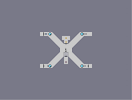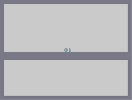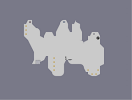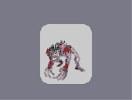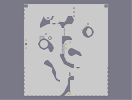X Marks the Spot Test Castle of Stone Glory Small Cave ghoul Abstract

Amazing. Loved how fire is rendered.

Fucking.
Shit.

Fucking.
Shit.

### Holy.

Fucking.
Shit.
Superb. Great effect.

!

AWESOME. 5/5

### by far one of the best

narts ive ever seen
5aved

### YOU

must get this 1 million times a day, but

WOW!!!! 5/5 THIS IS AMAZING YOU ROCK!

### This is one of those maps

that make me say "holy crap" when I first see it. out loud.
Maybe it's not flawless, but nothing is "flawless," not even ]{NO3's insane art. Deserves a 5.

### hmmm

2 things:
1. how do u know its 5 less objects and 2. add 5 objects :)

easily

### You commented to my map

Saying: the empty spots should have been filled and the bubbles look flat.

You think the same as I do. ^^ Agreed 100%.
Thanks for the constructive comment ^^.

WOW!
5/5

5aved

### well done

nice colours. the bricks are a bit warped in places.
but I don't feel it is worthy of a five... 4 chould do nicely...

### I must be deluded

But I dont see how this is messy at all. All I saw was some great texture work and inventive colours.

Sloppy and messy. Apparently this kind of scenes shouldn't be rendered with N objects.

### Pawz

I guess thats why the Last Supper sucks :P

### Sloppy

equals the effect isn't as tight as it could have been with al ittle more care.

Afternoon Dragon looks ok at a glance, but look for more than that and it's a very weak art, imo.

### I don't agree

It's the full use of space that makes this so special for me, and with full use I don't mean like one object and a background, but objects that are all equally to eachother. And another thing that made it special: it's not a dragon Nart

### NR

I'm not going to vote on this and I hope to GOD it does not get featured.

This is nowhere near the awesomeness of AfternoonDragon IMO. Sure, they are both "sloppy" (I'm still not quite sure what that is supposed to mean, as any hand-made art can be called "sloppy" in one way or another) but this one has too much going on for me to think it is any good. I feel like SkyRay has made better Nart's than this, I'm afraid.

I guess since it's already done you probably feel proud. This does have a lot of rates, and though that's nice, I think if you were to do another Nart you should put the focus on a single idea or object or simple scenery, as this one seems overcrowded.

### CLARIE150:

Why'd you try to load it in NED? Isn't that not the point of the map?

One of the better N-arts I've seen, but not perfect.

### Not Exactly...

Not Exactly Good... I mean, my N Reality NED slowed own to like 768 MS/F before it finished. You could have used a little less for the same detail; stuff was drawn and redrawn as another item covered it.
Still, it looks awesome from the thumbnail.

### woah

The shading on the bricks almost makes it look Photoshopped autogenerated, but looking at it closer, shows it's obviously not. Nice work!

btw, to the people who are saying it doesn't look as good close up... well, that's the point. Of course it won't. It's made up of N objects, not pixels. It's meant to look good in the thumbnail

### Ahhh,

Looks pretty awesome from the thumbnail, but close up is just ok. 4/5

### 4

don't really know what to say, but i do not find this amazing

i might...

### OH BEATRICE!!!

THAT IS AMAZING
5aved for sure, nice.

### i

love it. that is a really good n-art. 5aved it

### hmm...

it could use more detail... mabye make this V1? close up, it isnt that great... retouch it.

### Yoda, eganic now is.

Stolen all the attention from this map [nmaps.net] you have.

### wait!

how can you see me?

on noes!!!

lawl lawl lawl

### Meh

my vote brought it down. sorry.
I don't think it's 'flawless', so it didn't get a five.

I like the thunder the best. The rest of it is above average, but there's nothing really amazing about it. I do like the attention to detail with the bricks, though.
4

### this is awesome

it must have taken forever.

### Hah

several hours and this is still top of hot maps. Btw, eganic's avvy looks stupid now...

### its eh

the bellow the top of the wall was great, but the above the top of the wall needs to be sharper 4

### The epicness...

IT BURNS!!!! 500000000000000/5